• 贝叶斯分类，主要讲述高斯贝叶斯的分类方法和如何分类。
• ## 带你搞懂朴素贝叶斯分类算法

万次阅读 多人点赞 2017-04-14 16:19:33
贝叶斯分类是一类分类算法的总称，这类算法均以贝叶斯定理为基础，故统称为贝叶斯分类。而朴素朴素贝叶斯分类贝叶斯分类中最简单，也是常见的一种分类方法。这篇文章我尽可能用直白的话语总结一下我们学习会上讲到...
最新人工智能论文：http://paperreading.club

带你搞懂朴素贝叶斯分类算

贝叶斯分类是一类分类算法的总称，这类算法均以贝叶斯定理为基础，故统称为贝叶斯分类。而朴素朴素贝叶斯分类是贝叶斯分类中最简单，也是常见的一种分类方法。这篇文章我尽可能用直白的话语总结一下我们学习会上讲到的朴素贝叶斯分类算法，希望有利于他人理解。

1  分类问题综述

对于分类问题，其实谁都不会陌生，日常生活中我们每天都进行着分类过程。例如，当你看到一个人，你的脑子下意识判断他是学生还是社会上的人；你可能经常会走在路上对身旁的朋友说“这个人一看就很有钱”之类的话，其实这就是一种分类操作。

既然是贝叶斯分类算法，那么分类的数学描述又是什么呢？

从数学角度来说，分类问题可做如下定义：已知集合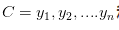和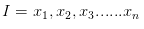，确定映射规则y = f(x)，使得任意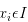有且仅有一个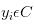,使得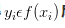成立。

其中C叫做类别集合，其中每一个元素是一个类别，而I叫做项集合（特征集合），其中每一个元素是一个待分类项，f叫做分类器。分类算法的任务就是构造分类器f。

分类算法的内容是要求给定特征，让我们得出类别，这也是所有分类问题的关键。那么如何由指定特征，得到我们最终的类别，也是我们下面要讲的，每一个不同的分类算法，对应着不同的核心思想。

本篇文章，我会用一个具体实例，对朴素贝叶斯算法几乎所有的重要知识点进行讲解。

2  朴素贝叶斯分类

那么既然是朴素贝叶斯分类算法，它的核心算法又是什么呢？

是下面这个贝叶斯公式：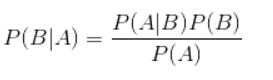换个表达形式就会明朗很多，如下：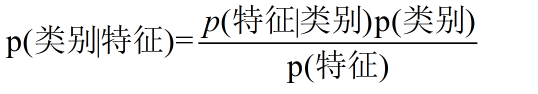我们最终求的p(类别|特征)即可！就相当于完成了我们的任务。

3  例题分析

下面我先给出例子问题。

给定数据如下：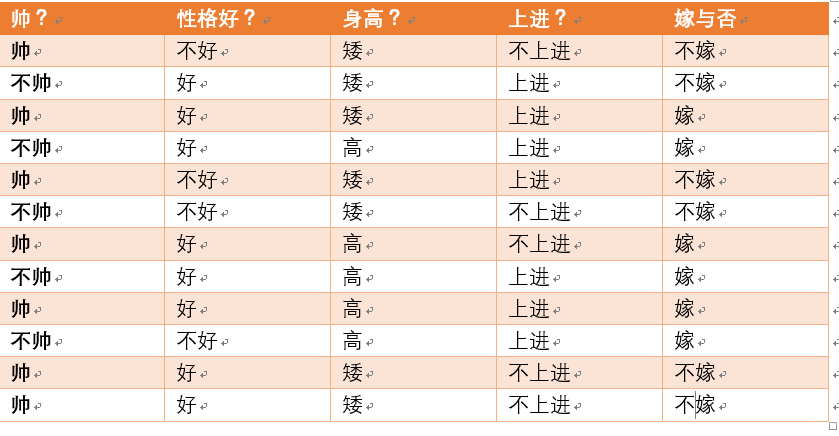现在给我们的问题是，如果一对男女朋友，男生想女生求婚，男生的四个特点分别是不帅，性格不好，身高矮，不上进，请你判断一下女生是嫁还是不嫁？

这是一个典型的分类问题，转为数学问题就是比较p(嫁|(不帅、性格不好、身高矮、不上进))与p(不嫁|(不帅、性格不好、身高矮、不上进))的概率，谁的概率大，我就能给出嫁或者不嫁的答案！

这里我们联系到朴素贝叶斯公式：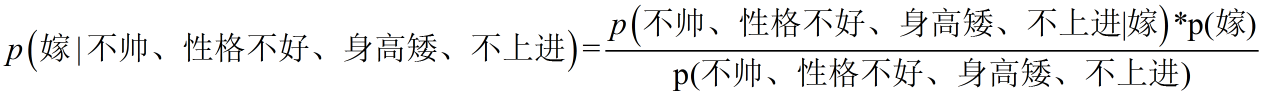我们需要求p(嫁|(不帅、性格不好、身高矮、不上进),这是我们不知道的，但是通过朴素贝叶斯公式可以转化为好求的三个量.

p(不帅、性格不好、身高矮、不上进|嫁)、p（不帅、性格不好、身高矮、不上进)、p(嫁)（至于为什么能求，后面会讲，那么就太好了，将待求的量转化为其它可求的值，这就相当于解决了我们的问题！）

4  朴素贝叶斯算法的朴素一词解释

那么这三个量是如何求得？

是根据已知训练数据统计得来，下面详细给出该例子的求解过程。

回忆一下我们要求的公式如下：那么我只要求得p(不帅、性格不好、身高矮、不上进|嫁)、p（不帅、性格不好、身高矮、不上进)、p(嫁)即可，好的，下面我分别求出这几个概率，最后一比，就得到最终结果。

p(不帅、性格不好、身高矮、不上进|嫁) = p(不帅|嫁)*p(性格不好|嫁)*p(身高矮|嫁)*p(不上进|嫁)，那么我就要分别统计后面几个概率，也就得到了左边的概率！

等等，为什么这个成立呢？学过概率论的同学可能有感觉了，这个等式成立的条件需要特征之间相互独立吧！

对的！这也就是为什么朴素贝叶斯分类有朴素一词的来源，朴素贝叶斯算法是假设各个特征之间相互独立，那么这个等式就成立了！

但是为什么需要假设特征之间相互独立呢？

1、我们这么想，假如没有这个假设，那么我们对右边这些概率的估计其实是不可做的，这么说，我们这个例子有4个特征，其中帅包括{帅，不帅}，性格包括{不好，好，爆好}，身高包括{高，矮，中}，上进包括{不上进，上进}，那么四个特征的联合概率分布总共是4维空间，总个数为2*3*3*2=36个。

36个，计算机扫描统计还可以，但是现实生活中，往往有非常多的特征，每一个特征的取值也是非常之多，那么通过统计来估计后面概率的值，变得几乎不可做，这也是为什么需要假设特征之间独立的原因。

2、假如我们没有假设特征之间相互独立，那么我们统计的时候，就需要在整个特征空间中去找，比如统计p(不帅、性格不好、身高矮、不上进|嫁),

我们就需要在嫁的条件下，去找四种特征全满足分别是不帅，性格不好，身高矮，不上进的人的个数，这样的话，由于数据的稀疏性，很容易统计到0的情况。 这样是不合适的。

根据上面俩个原因，朴素贝叶斯法对条件概率分布做了条件独立性的假设，由于这是一个较强的假设，朴素贝叶斯也由此得名！这一假设使得朴素贝叶斯法变得简单，但有时会牺牲一定的分类准确率。

好的，上面我解释了为什么可以拆成分开连乘形式。那么下面我们就开始求解！

我们将上面公式整理一下如下：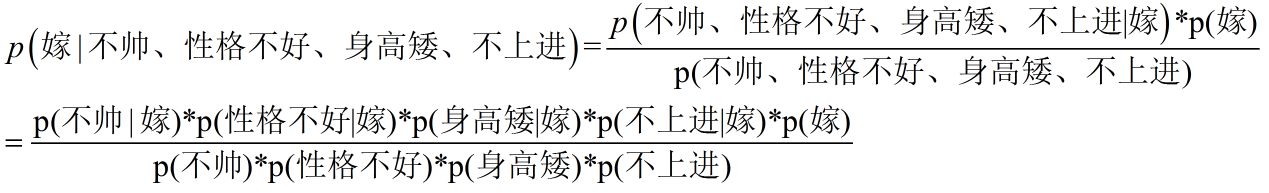下面我将一个一个的进行统计计算（在数据量很大的时候，根据中心极限定理，频率是等于概率的，这里只是一个例子，所以我就进行统计即可）。

p(嫁)=？

首先我们整理训练数据中，嫁的样本数如下：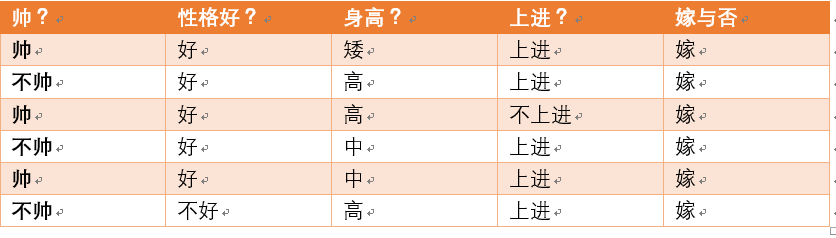则 p(嫁) = 6/12（总样本数） = 1/2

p(不帅|嫁)=？统计满足样本数如下：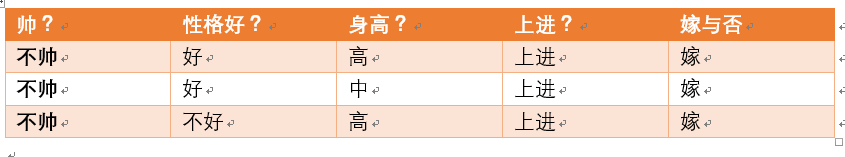则p(不帅|嫁) = 3/6 = 1/2 在嫁的条件下，看不帅有多少

p(性格不好|嫁)= ？统计满足样本数如下：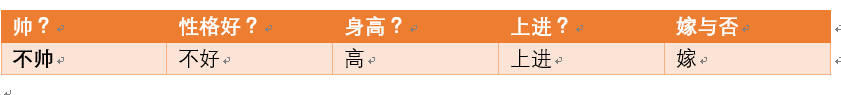则p(性格不好|嫁)= 1/6

p（矮|嫁） = ?统计满足样本数如下：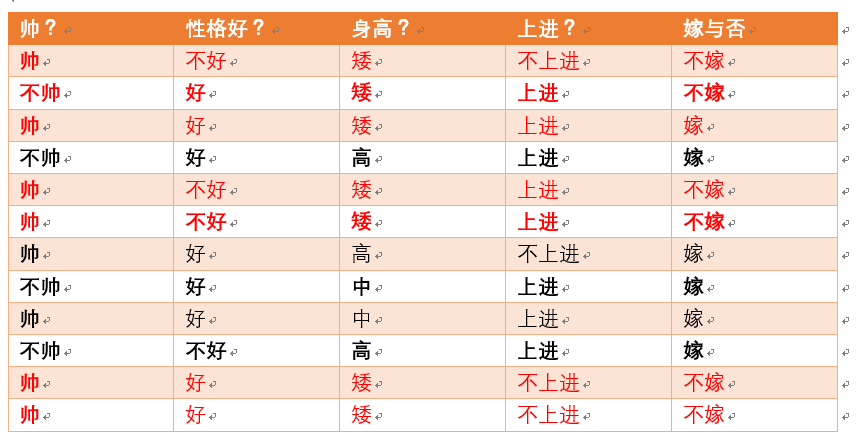则p(矮|嫁) = 1/6

p(不上进|嫁) = ?统计满足样本数如下：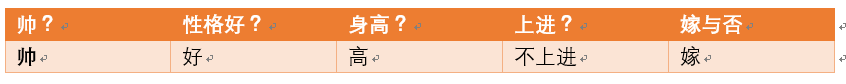则p(不上进|嫁) = 1/6

下面开始求分母，p(不帅)，p（性格不好），p（矮），p（不上进）

统计样本如下：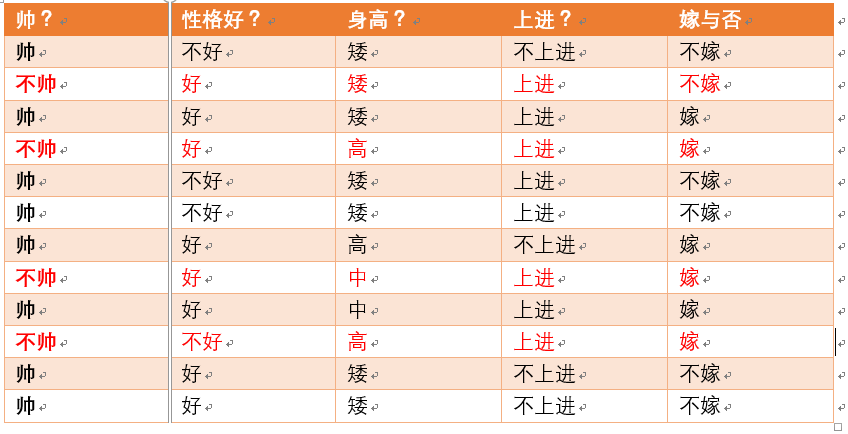不帅统计如上红色所示，占4个，那么p（不帅） = 4/12 = 1/3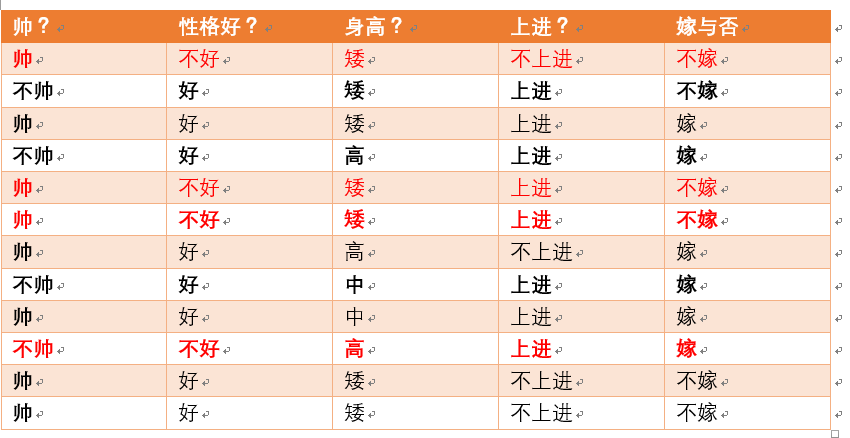性格不好统计如上红色所示，占4个，那么p（性格不好） = 4/12 = 1/3身高矮统计如上红色所示，占7个，那么p（身高矮） = 7/12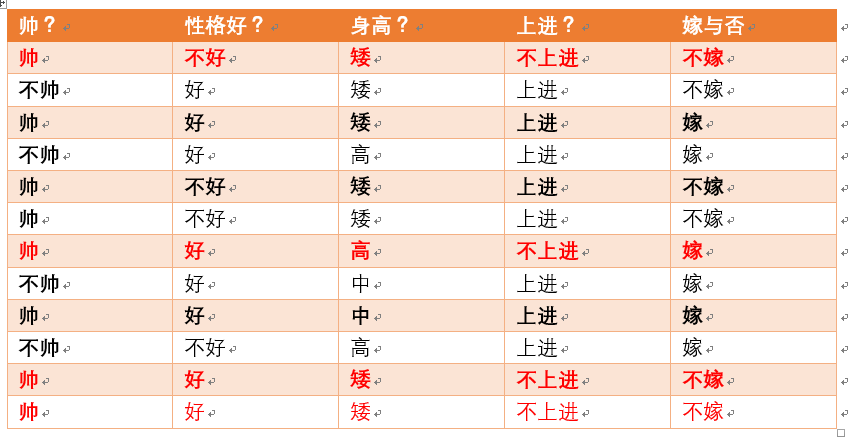不上进统计如上红色所示，占4个，那么p（不上进） = 4/12 = 1/3

到这里，要求p(不帅、性格不好、身高矮、不上进|嫁)的所需项全部求出来了，下面我带入进去即可，= (1/2*1/6*1/6*1/6*1/2)/(1/3*1/3*7/12*1/3)

下面我们根据同样的方法来求p(不嫁|不帅，性格不好，身高矮，不上进)，完全一样的做法，为了方便理解，我这里也走一遍帮助理解。首先公式如下：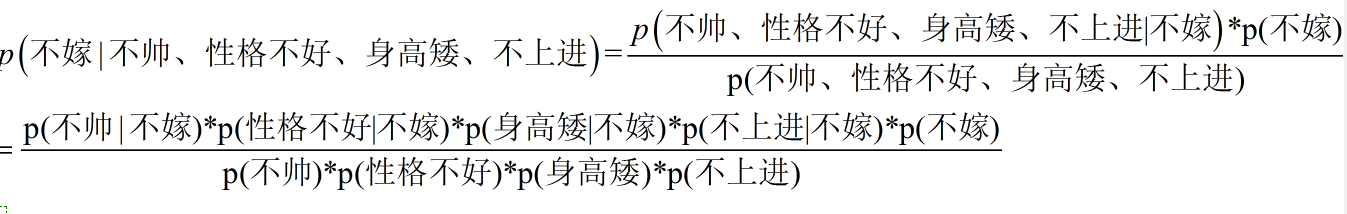下面我也一个一个来进行统计计算，这里与上面公式中，分母是一样的，于是我们分母不需要重新统计计算！

p（不嫁）=？根据统计计算如下（红色为满足条件）：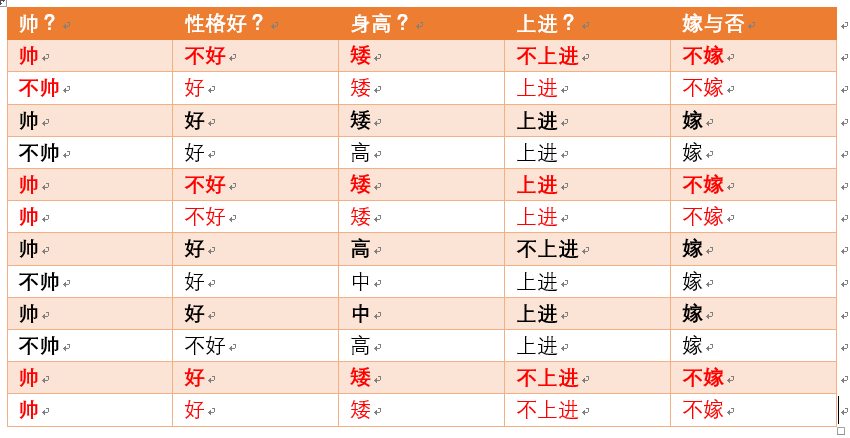则p(不嫁)=6/12 = 1/2

p(不帅|不嫁) = ？统计满足条件的样本如下（红色为满足条件）：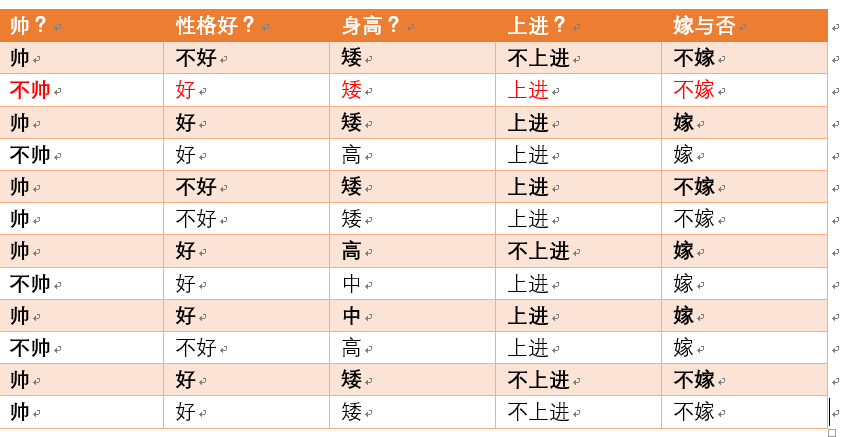则p（不帅|不嫁） = 1/6

p（性格不好|不嫁） = ？据统计计算如下（红色为满足条件）：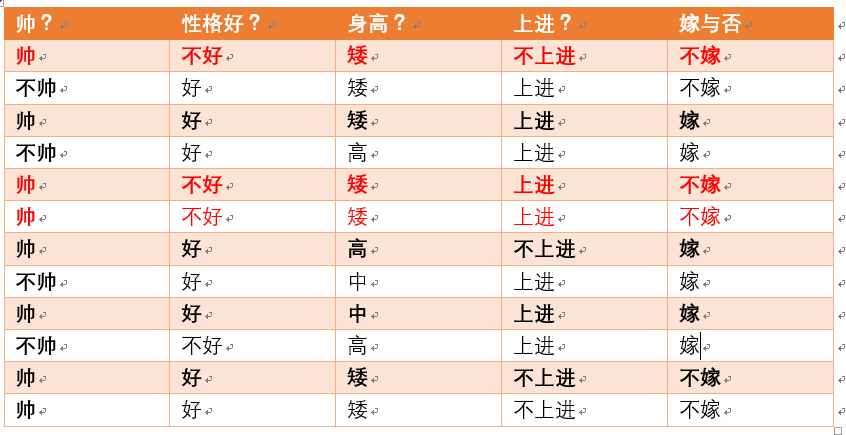则p（性格不好|不嫁） =3/6 = 1/2

p（矮|不嫁） = ？据统计计算如下（红色为满足条件）：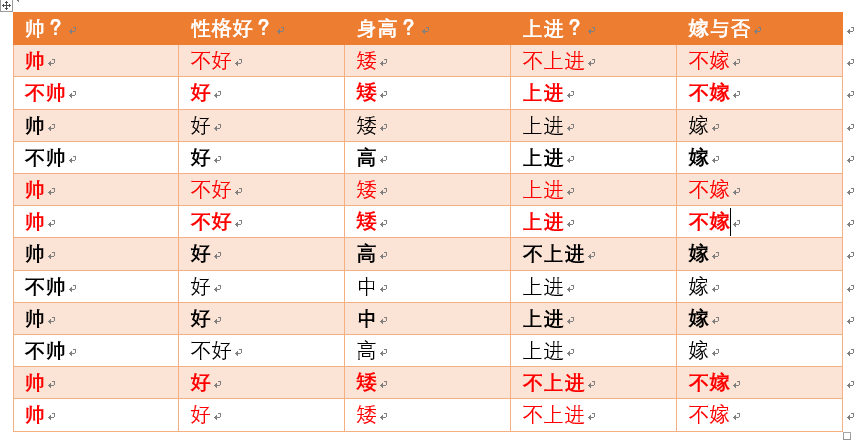则p（矮|不嫁） = 6/6 = 1

p（不上进|不嫁） = ？据统计计算如下（红色为满足条件）：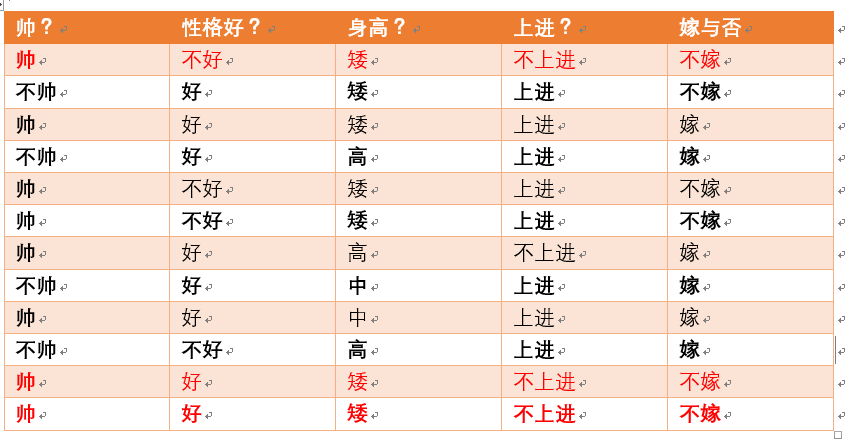则p（不上进|不嫁） = 3/6 = 1/2

那么根据公式：p (不嫁|不帅、性格不好、身高矮、不上进) = ((1/6*1/2*1*1/2)*1/2)/(1/3*1/3*7/12*1/3)

很显然(1/6*1/2*1*1/2) > (1/2*1/6*1/6*1/6*1/2)

于是有p (不嫁|不帅、性格不好、身高矮、不上进)>p (嫁|不帅、性格不好、身高矮、不上进)

所以我们根据朴素贝叶斯算法可以给这个女生答案，是不嫁！！！！

5  朴素贝叶斯分类的优缺点

优点：

（1） 算法逻辑简单,易于实现（算法思路很简单，只要使用贝叶斯公式转化医学即可！）

（2）分类过程中时空开销小（假设特征相互独立，只会涉及到二维存储）

缺点：

理论上，朴素贝叶斯模型与其他分类方法相比具有最小的误差率。但是实际上并非总是如此，这是因为朴素贝叶斯模型假设属性之间相互独立，这个假设在实际应用中往往是不成立的，在属性个数比较多或者属性之间相关性较大时，分类效果不好。

而在属性相关性较小时，朴素贝叶斯性能最为良好。对于这一点，有半朴素贝叶斯之类的算法通过考虑部分关联性适度改进。

整个例子详细的讲解了朴素贝叶斯算法的分类过程，希望对大家的理解有帮助~

参考：李航博士《统计学习方法》

算法杂货铺--分类算法之朴素贝叶斯分类(Naive Bayesian classification)

致谢：德川，皓宇，继豪，施琦

原文地址：https://mp.weixin.qq.com/s?__biz=MzI4MDYzNzg4Mw==&mid=2247483819&idx=1&sn=7f1859c0a00248a4c658fa65f846f341&chksm=ebb4397fdcc3b06933816770b928355eb9119c4c80a1148b92a42dc3c08de5098fd6f278e61e#rd

展开全文• 朴素贝叶斯（Naive Bayes）原理+编程实现拉普拉斯修正的朴素贝叶斯分类器，以西瓜数据集3.0为训练集，对“测1”样本进行判别。
贝叶斯方法与朴素贝叶斯1.生成模型与判别模型2.贝叶斯2.1贝叶斯公式2.2贝叶斯方法3朴素贝叶斯3.1条件独立性假设3.2朴素贝叶斯Naive在何处？3.3朴素贝叶斯的三种模型3.4平滑技术3.5朴素贝叶斯优缺点3.6朴素贝叶斯的应用与代码实现
1.生成模型与判别模型
在概率生成模型(Probabilistic Generative Model)与朴素贝叶斯(Naive Bayes)中大概学习了一下概率生成模型以及朴素贝叶斯，今天在这里再总结一下生成模型与判别模型。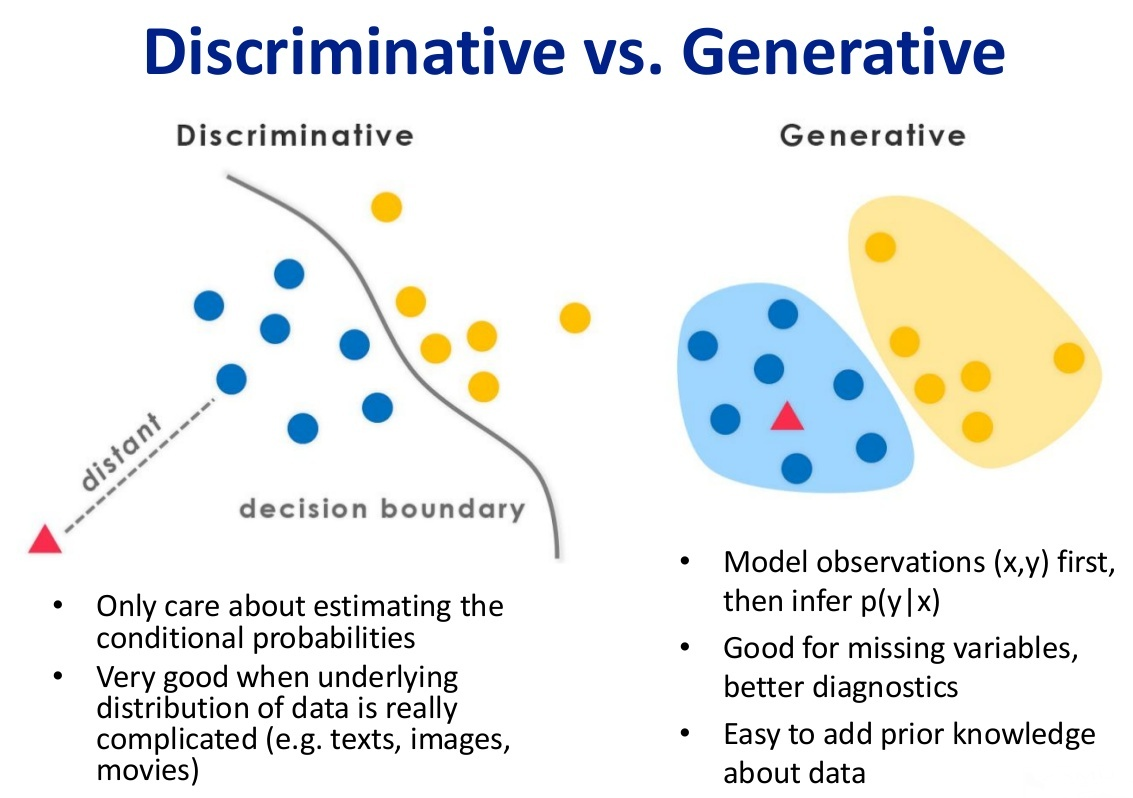上面这张图可以清楚的解释二者的差别。前面我们讲到， 所谓的机器学习模型，本质上是一个函数，其作用是实现从一个样本 $x$ 到样本的标记值 $y$ 的映射，即 $f(x;\theta ^{*})\rightarrow y$，或者求得标签的条件概率：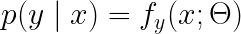例如有C1以及C2两种类别，我们需要判断样本属于哪一个class：

判别式模型：要确定一个样本是属于C1还是C2，用判别模型的方法是从历史数据中学习到模型，然后通过提取该样本的特征来预测出该样本属于C1的概率以及C2的概率。
生成式模型：根据C1的特征首先学习出一个C1的模型，然后根据C2的特征学习出一个C2的模型，然后从该样本中提取特征，放到C1模型中看概率是多少，然后再放到C2模型中看概率是多少，哪个大就是哪个。
判别模型之所以称为判别模型，就是因为它只是简单地判别就好了。根据样本X来判别它所属的类别，直接就求出了$p(y|x)$的大小
而生成模型，在概率生成模型(Probabilistic Generative Model)与朴素贝叶斯(Naive Bayes)中我们可以看到，我们要求：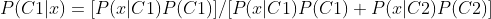P(C1)与P(C2)很好求，我们要求的是P(x|C1)以及P(x|C2)，也就是说我们要先求得C1与C2的具体分布，也就是上面说的，分别根据C1与C2的特征学得两个模型，得到模型之后代入，若P(C1|x)>0.5那x就属于C1，否则属于C2。

2.贝叶斯
2.1贝叶斯公式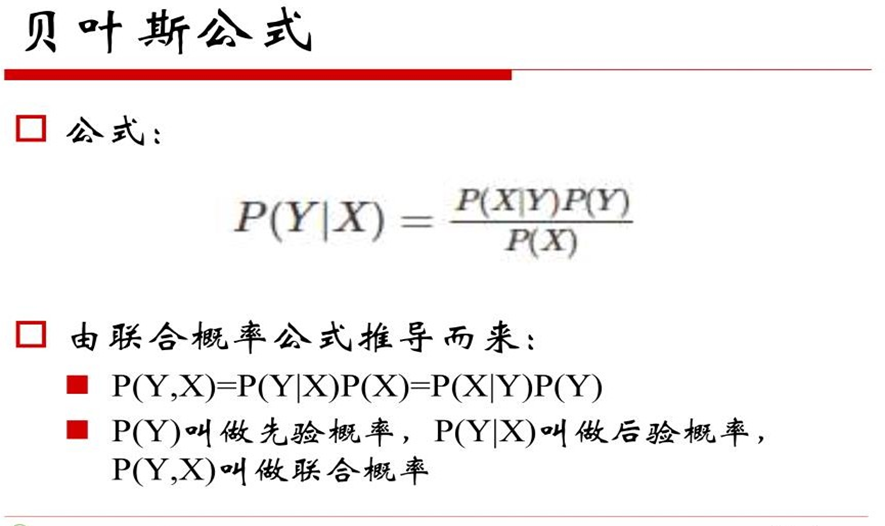学过概率论的都应该对上面这个公式很熟悉，这里就不再细讲了。这里需要注意的点是注意区分先验概率、后验概率、联合概率三个概念。
2.2贝叶斯方法
机器学习的最终目的就是回归 or 分类，这里二者都可以理解为预测，回归很好理解，分类也可以理解为预测属于某一类的概率是多少。 我们把上述贝叶斯公式中的X理解为“具有某特征”，把Y理解成“类别标签”，那么贝叶斯公式就可以表示为：
P(“属于某类”|“具有某特征”)=P(“具有某特征”|“属于某类”)P(“属于某类”)/P(“具有某特征”)。
贝叶斯方法把计算“具有某特征条件下属于某类（就是分类）”的概率转化为需要计算“属于某类条件下具有某特征（分别训练模型）”的概率，属于有监督学习。
3朴素贝叶斯
我们以垃圾邮件识别来引出朴素贝叶斯。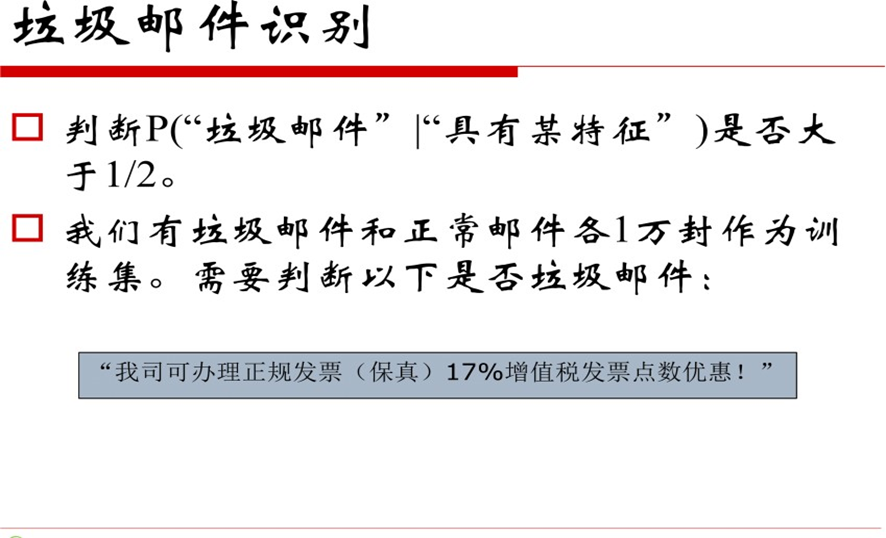我们要做的是判断上面那个邮件：“我司可办理正规发票（保真）17%增值税发票点数优惠”是否属于垃圾邮件。我们不能直接判断一整个邮件是否属于垃圾邮件，我们首先想到的应该是“分词”，所谓分词就是把邮件拆成一个个的词语，通过该词语是否属于训练样本中的垃圾邮件来做出进一步判断：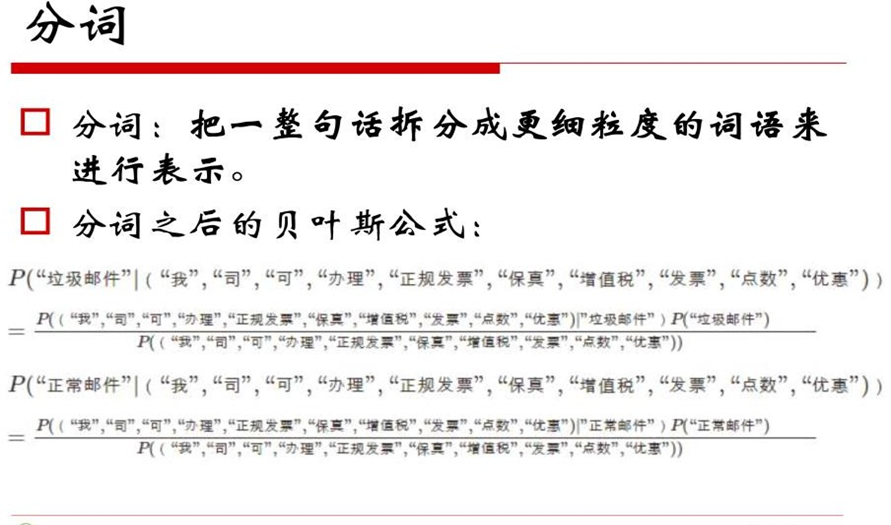前面我们提到：
贝叶斯方法把计算“具有某特征条件下属于某类（就是分类）”的概率转化为需要计算“属于某类条件下具有某特征（分别训练模型）”的概率，属于有监督学习。 也就是说，我们现在要计算的是：正常邮件 or 垃圾邮件中具有上述那些词语的概率。
3.1条件独立性假设
引入条件独立假设：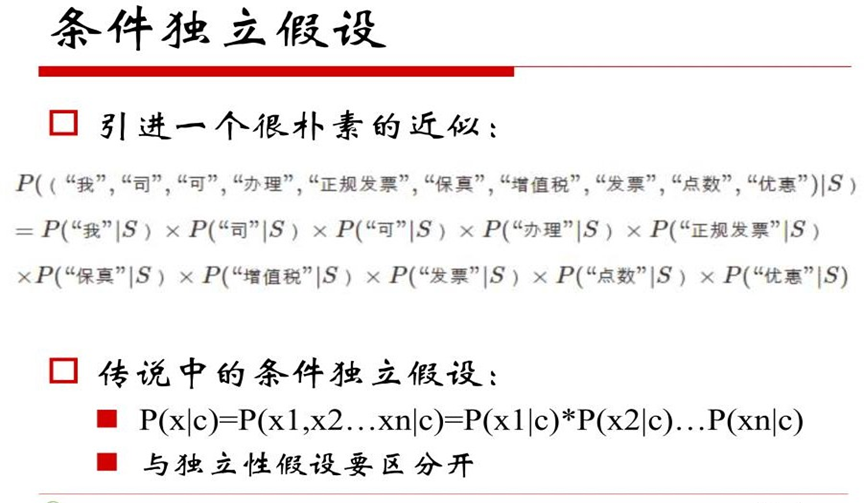我们把求某一类邮件中包含上述那些词语的概率等同于某一类邮件中包含每一种词语概率的乘积！！这其实就是朴素贝叶斯的实质，也是条件独立假设的实质。
既然如此，那每一项都变得特别好求解了：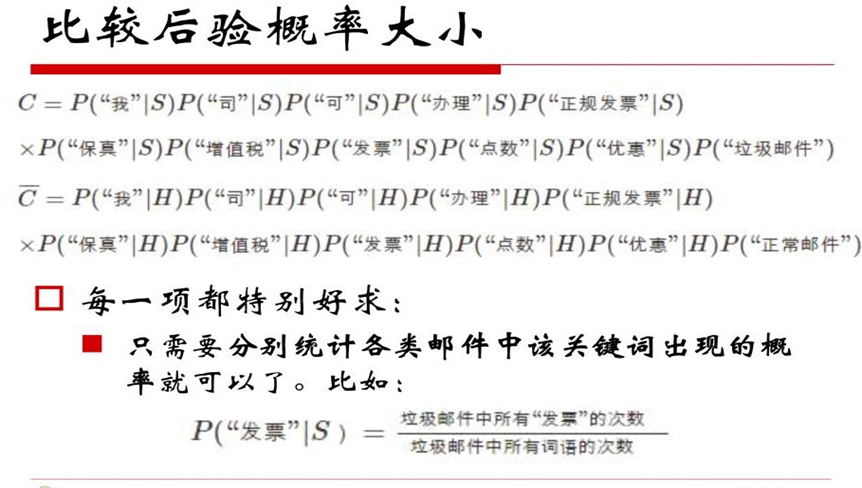要算P(x1|C)，那就直接在训练集中统计一下，看看x1在类别c1中出现的概率是多少即可。
3.2朴素贝叶斯Naive在何处？

加上条件独立假设的贝叶斯方法就是朴素贝叶斯方法（Naive Bayes）
由于乘法交换律，朴素贝叶斯中算出来交换词语顺序的条件概率完全一样

上述2的意思是：对于朴素贝叶斯模型来讲，“我司可办理正规发票”与“正规发票可办理我司”是一样的，会给出相同的判别结果，这点应该很好理解，因为你有了条件独立假设，abc与cba肯定大小是一样的，自然概率也是一样的，判别结果也就一样了。
3.3朴素贝叶斯的三种模型

多项式模型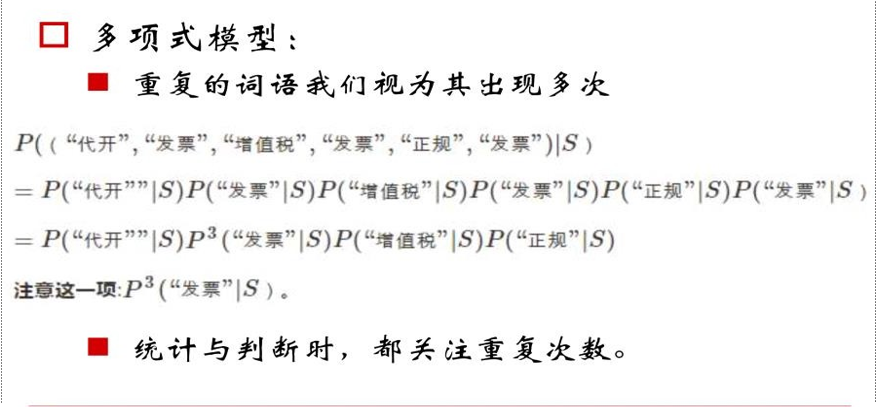多项式模型跟我们思维是一样的，例如在上述条件概率中，“发票”一词语出现了三次，那利用条件独立假设条件之后，自然应该出现三次方，这就是多项式模型。

伯努利模型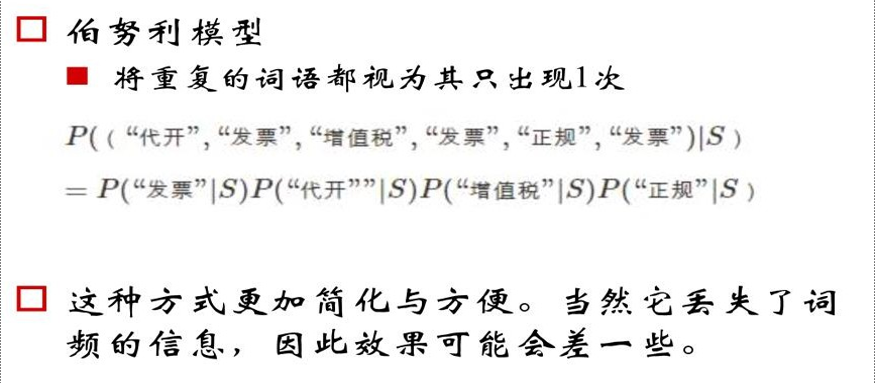在伯努利模型中我们可以看到，即使“发票”一词出现三次，但是我们在最终计算时只算一次。

混合模型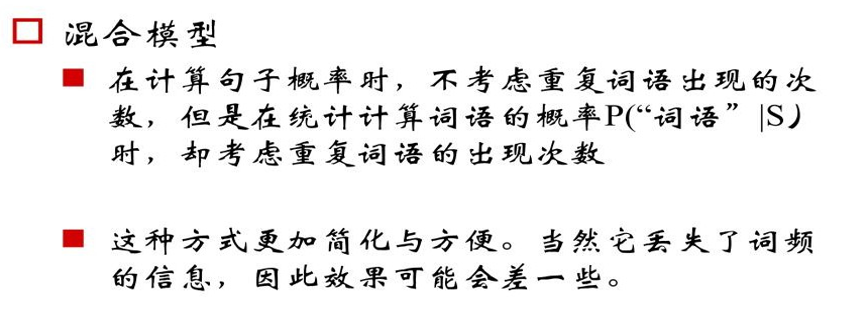在混合模型中，我们计算句子概率时不考虑重复词语出现的次数，但在统计计算词语概率时考虑重复次数。
一张图概括三种模型：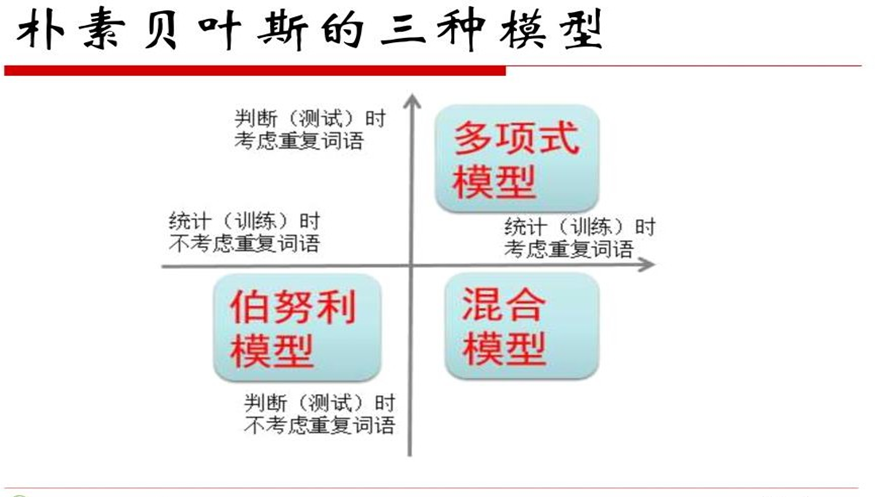3.4平滑技术
我们先来看这样一种可能：我们在求P(“正规发票”|S)时，通常的做法就是在训练集中遍历，看看“正规发票”一词出现了多少次，但是好巧不巧，该词在训练集中一次都没有出现过，那概率不就是0了么，然后条件独立假设相乘。。。于是整个概率都变成了0，这显然是极不合理的，但是这种情况却不少见。
于是我们引入了平滑技术这一概念来解决这个问题：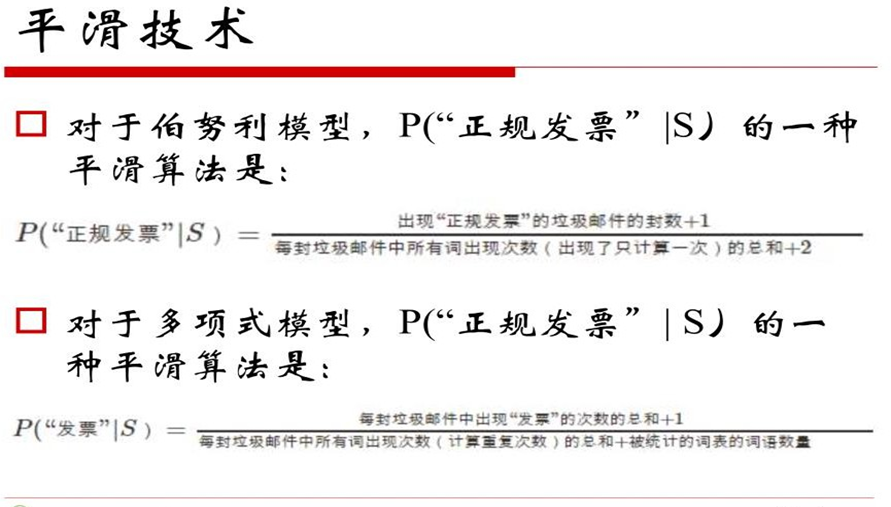这样，上述概率就不为0了，方便后续判断。
3.5朴素贝叶斯优缺点
优点：

对待预测样本进行预测，过程简单速度快
对于多分类问题也同样有效，复杂度也不会有大程度地上升。
在分布独立这个假设成立的情况下，贝叶斯的分类效果很好，会略胜于逻辑回归，我们需要的样本量也更少一点。
对于类别类的输入特征变量，效果非常好。对于数值型变量特征，我们默认它符合正态分布。

缺点：

如果测试集中的一个类别变量特征在训练集里面没有出现过，那么概率就是0，预测功能就将失效，平滑技术可以解决这个问题
朴素贝叶斯中有分布独立的假设前提，但是在现实生活中，这个条件很难满足。

3.6朴素贝叶斯的应用与代码实现
作业：编程实现拉普拉斯修正的朴素贝叶斯分类器，并以西瓜数据集3.0为训练集，对“测1”样本进行判别。
数据集长这样：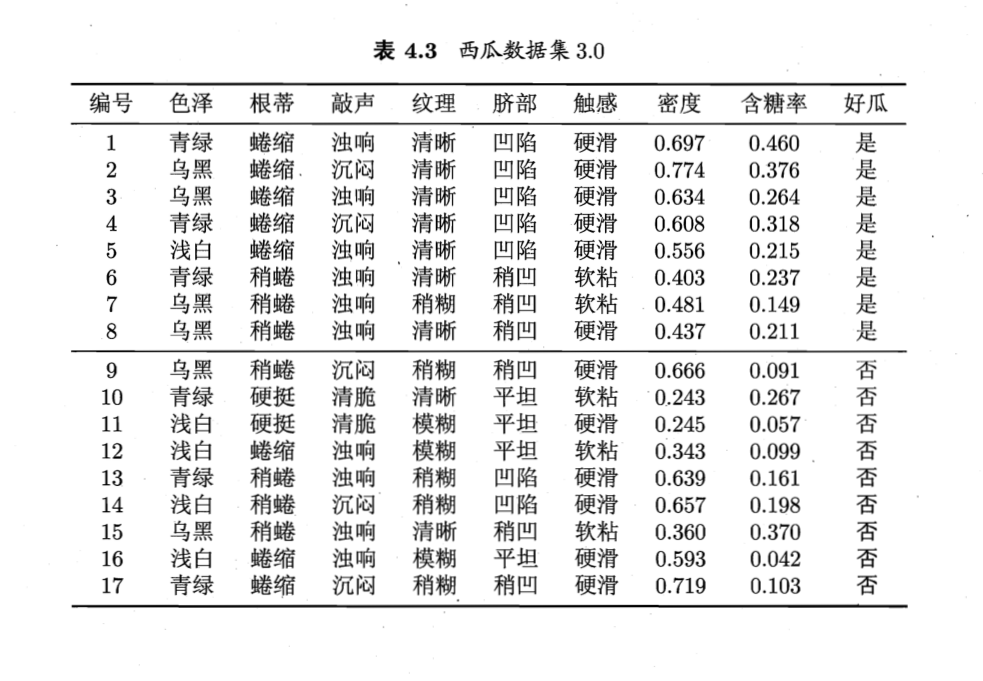代码实现：
import pandas as pd

datasets = pd.read_csv(r'E:\Program Files\PyCharm 2019.2\machinelearning\homework\西瓜3.0.csv', encoding="gbk")
del datasets['编号']
del datasets['好瓜']
return datasets

def NaiveBayes(test):
data = data.values.tolist()
for i in range(len(data)):
if data[i] == 1:
goodMelon.append(data[i])
else:
# 计算p(x|C1)=p1与p(x|C2)=p2
p1 = 1.0;p2 = 1.0
for j in range(len(test)):
x=0.0
for k in range(len(goodMelon)):
if goodMelon[k][j] == test[j]:
x = x + 1.0
p1 = p1 * ((x + 1.0) / (len(goodMelon) + 2.0))  # 拉普拉斯平滑
for j in range(len(test)):
x=0.0
x = x + 1.0
p2 = p2 * ((x + 1.0) / (len(badMelon) + 2.0))  # 拉普拉斯平滑
pc1 = len(goodMelon) / len(data)
pc2 = 1 - pc1
#贝叶斯公式
print('好瓜')
else:
print('坏瓜')

if __name__ == '__main__':
test=['青绿','蜷缩','浊响','清晰','凹陷','硬滑',0.697,0.460]
NaiveBayes(test)


结果：好瓜，分类正确。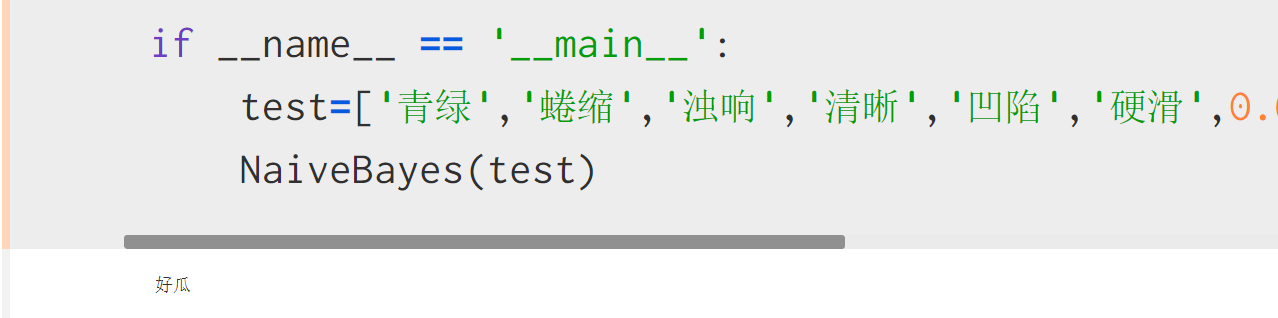展开全文机器学习
• MATLAB 贝叶斯分类MATLAB 贝叶斯分类MATLAB 贝叶斯分类MATLAB 贝叶斯分类
• 贝叶斯分类器理论知识。包括贝叶斯决策论，朴素贝叶斯分类器，半朴素贝叶斯分类器。
学习贝叶斯分类器，理论。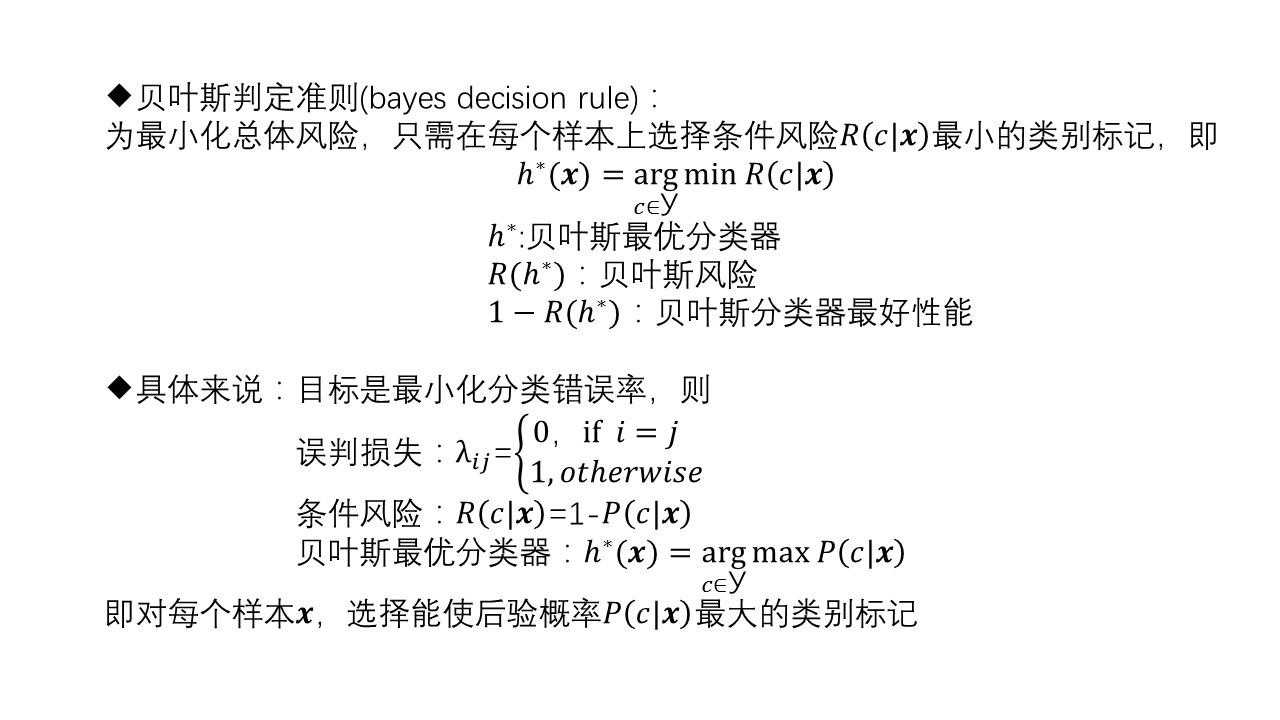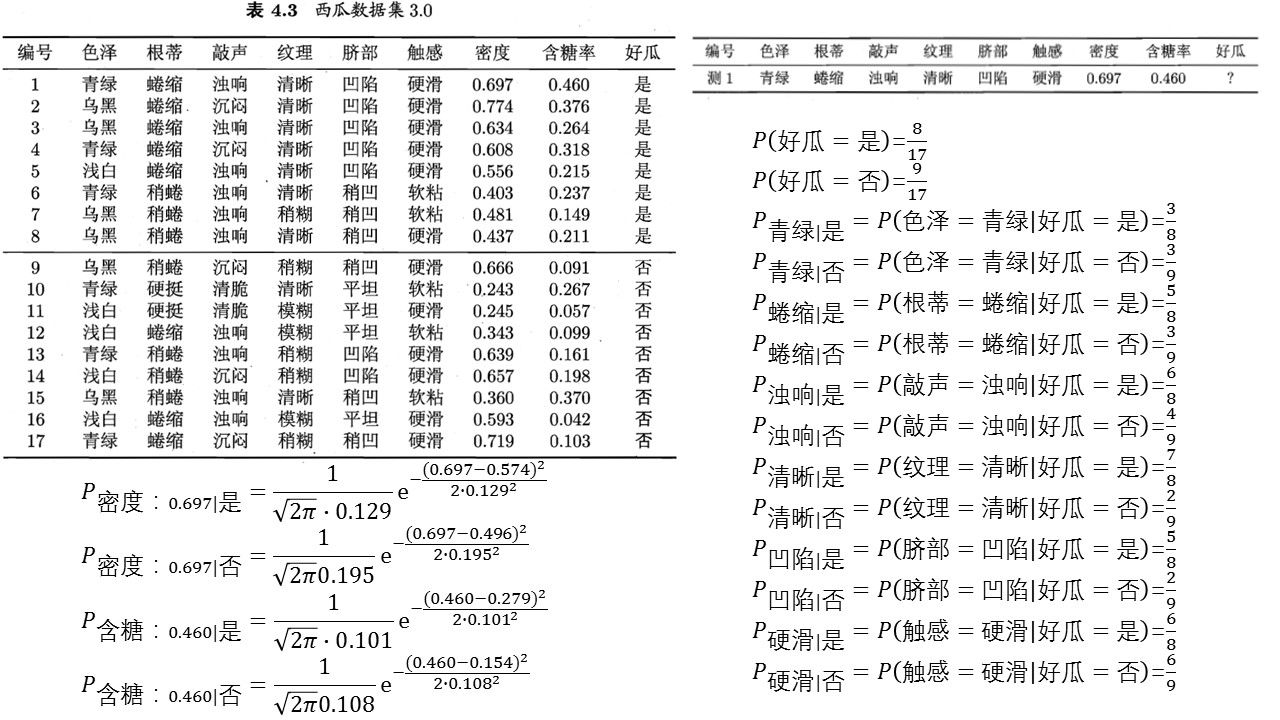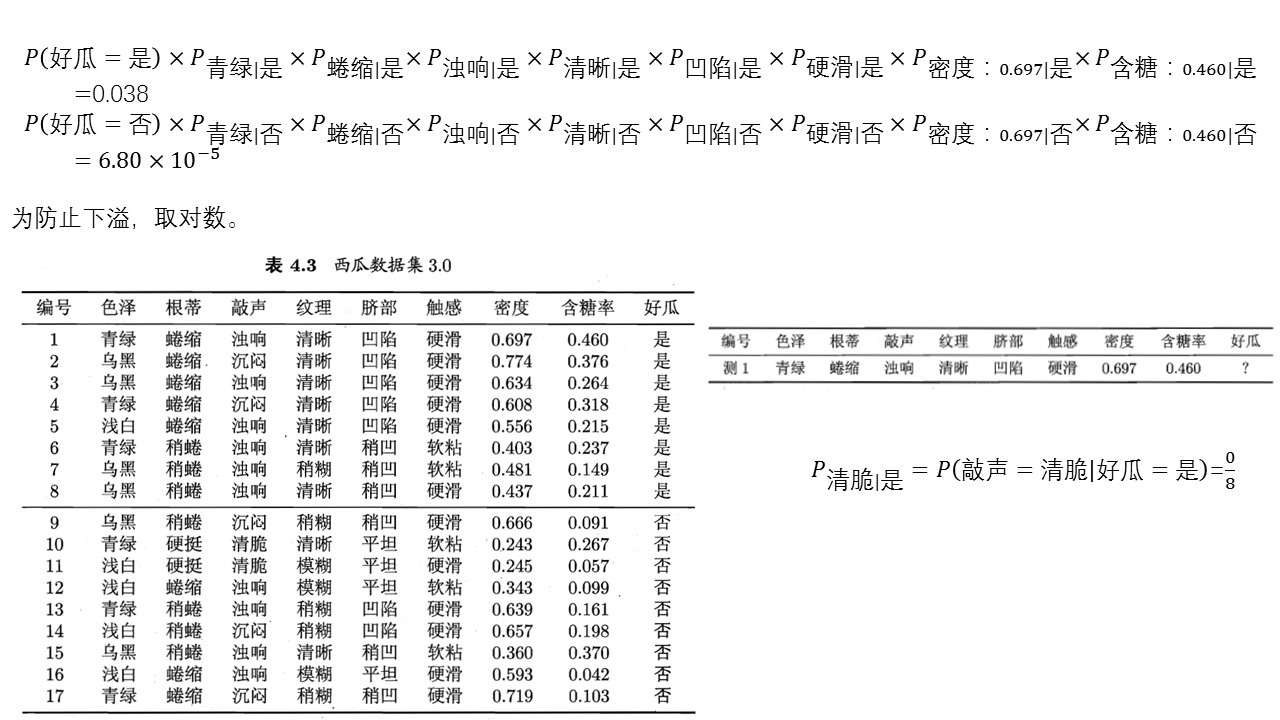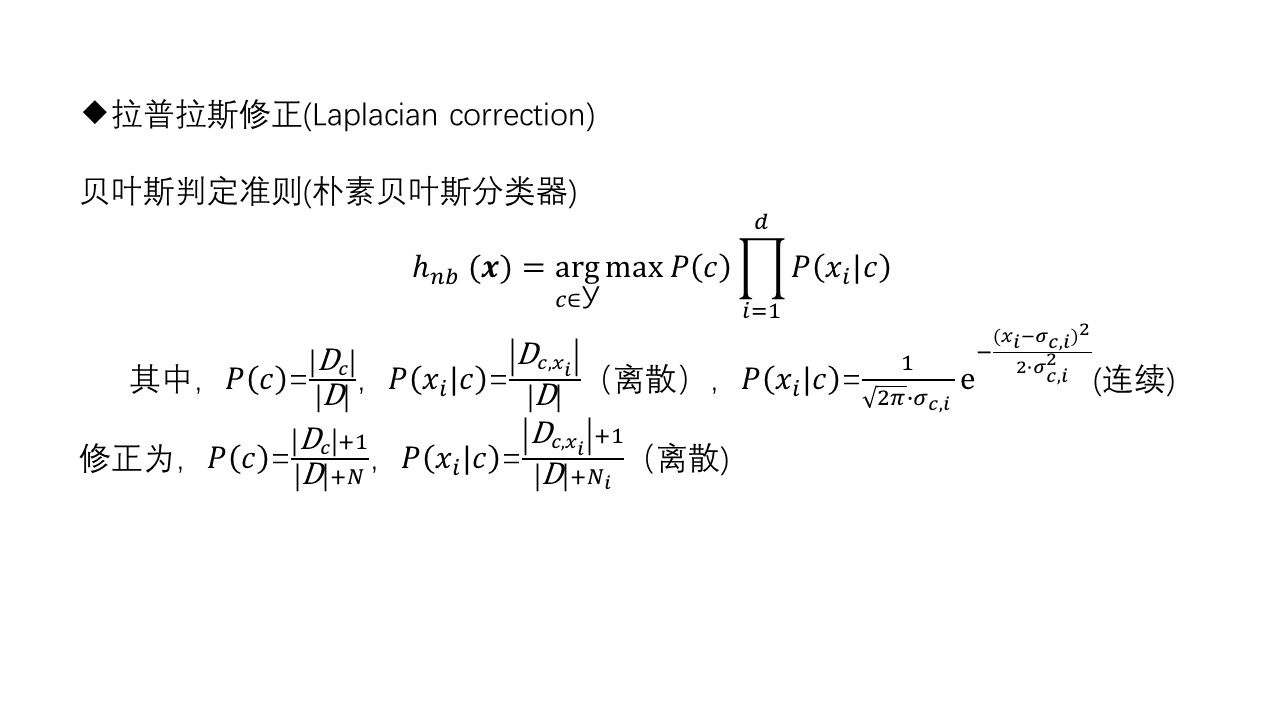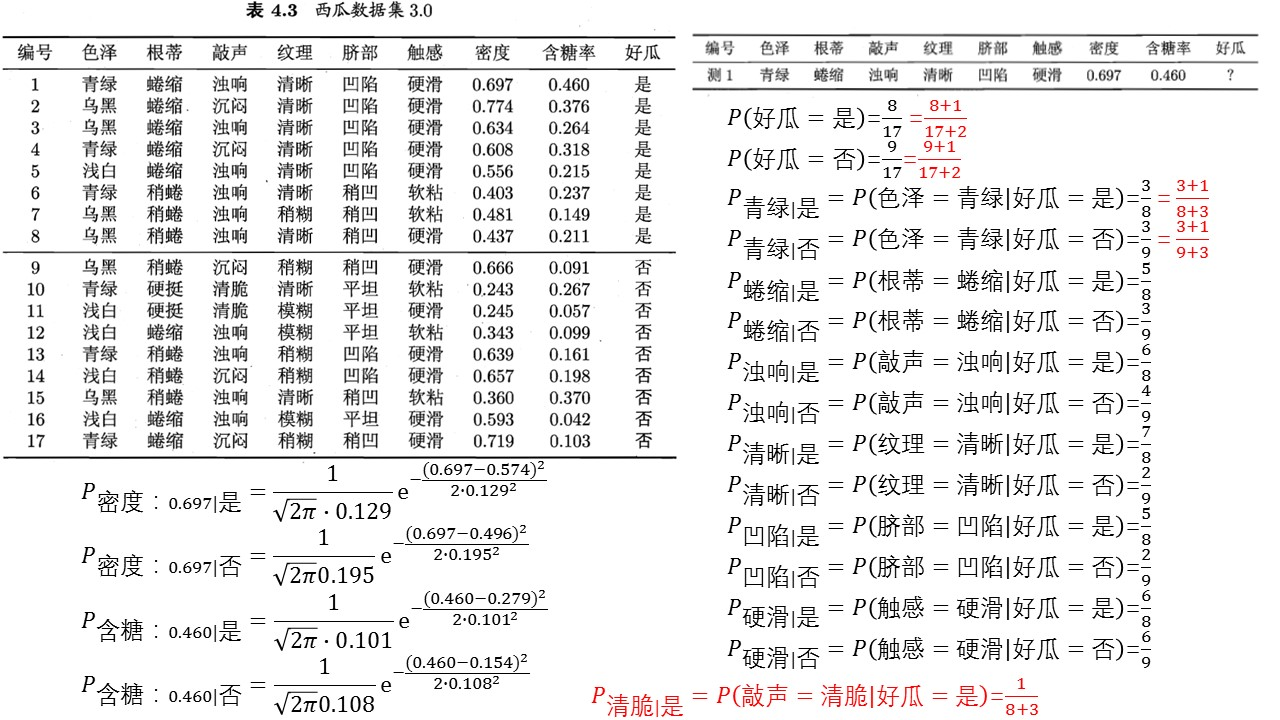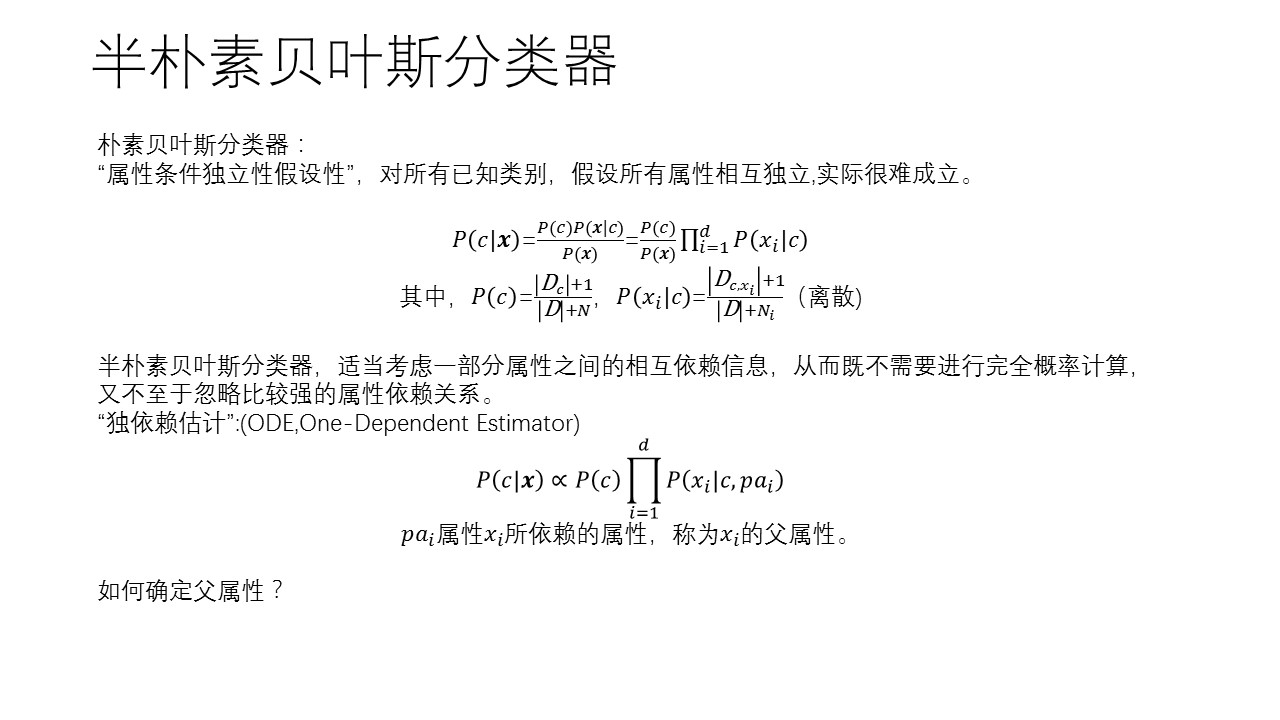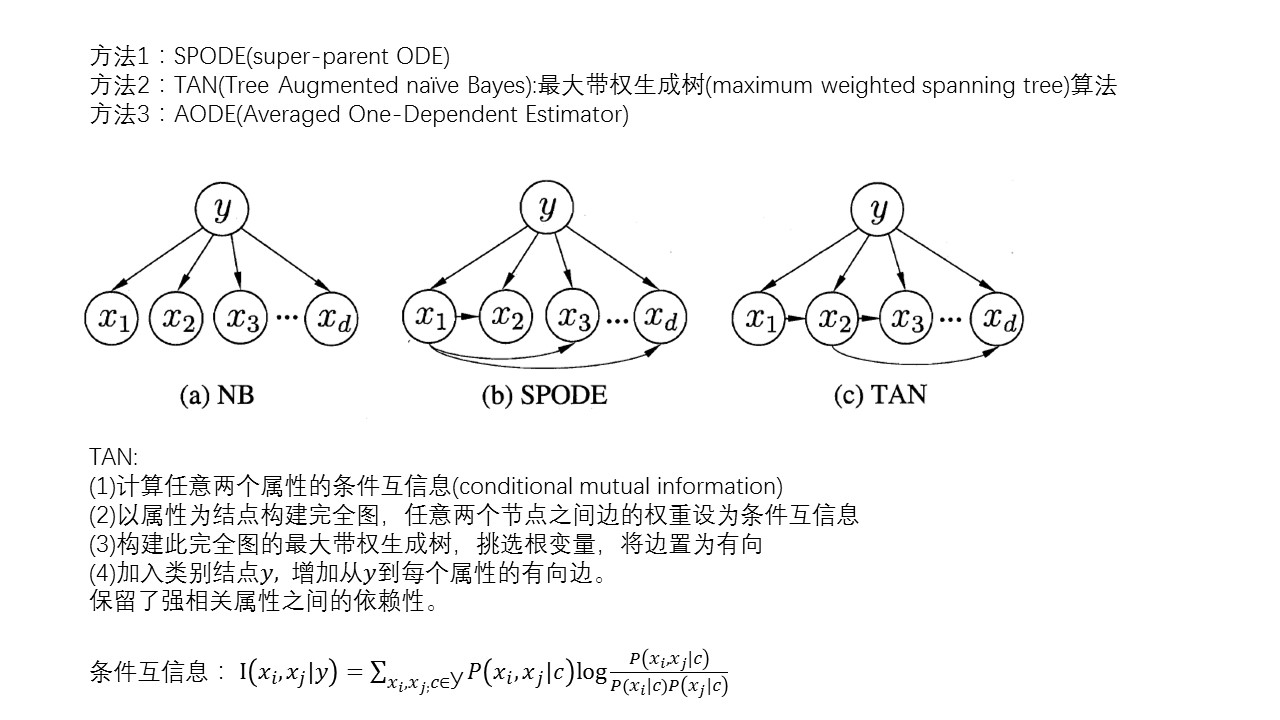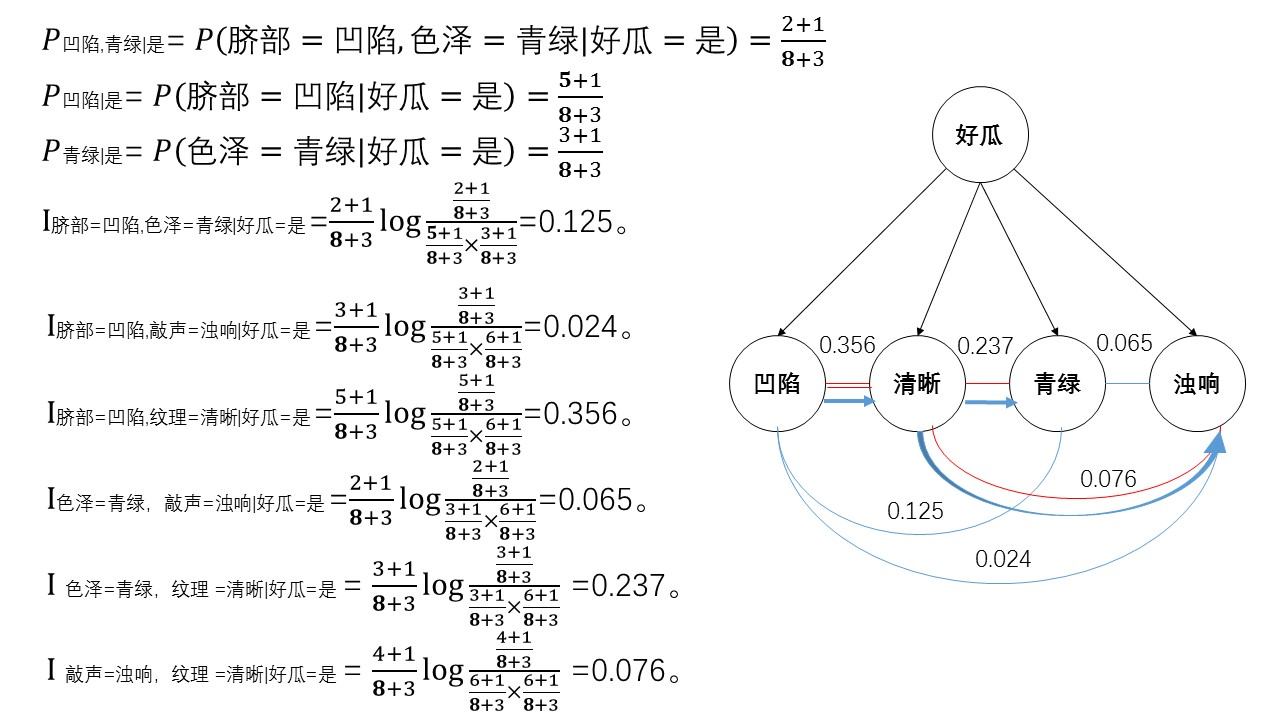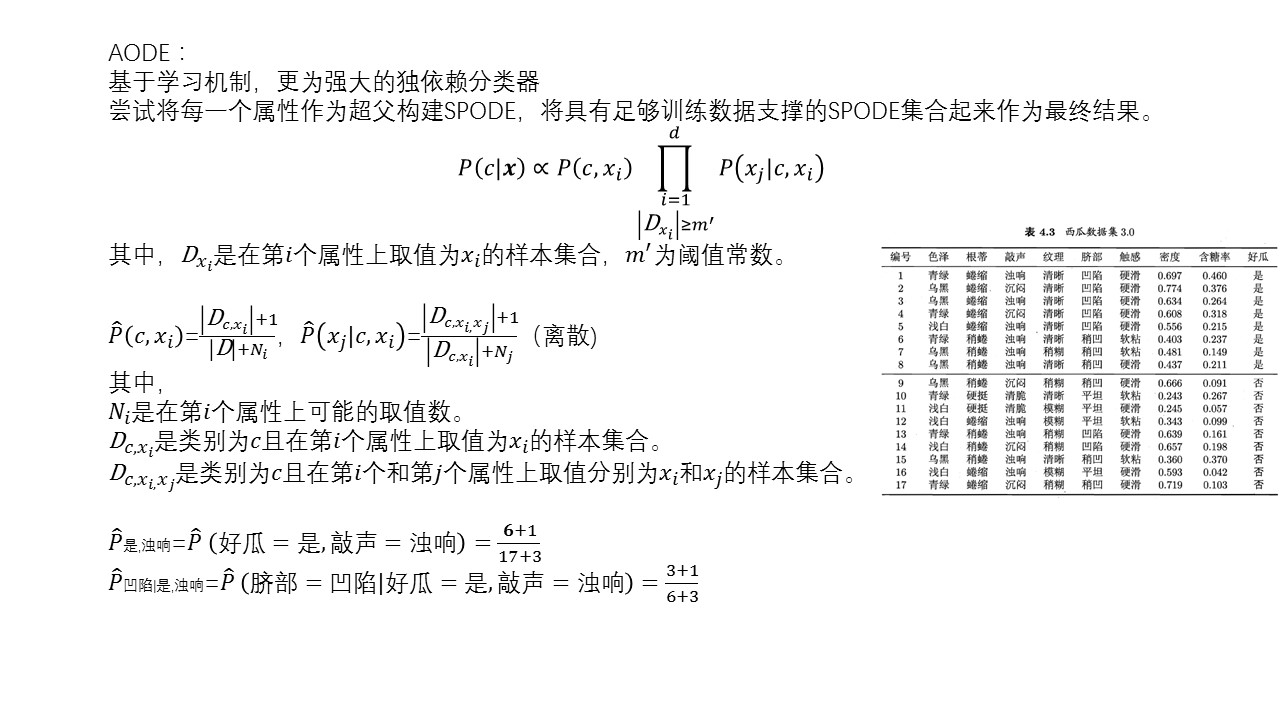展开全文• 贝叶斯分类是一类分类算法的总称，这类算法均以贝叶斯定理为基础，故统称为贝叶斯分类。本文作为分类算法的第一篇，将首先介绍分类问题，对分类问题进行一个正式的定义。然后，介绍贝叶斯分类算法的基础——贝叶斯...
• 贝叶斯分类算法是一大类分类算法的总称 贝叶斯分类算法以样本可能属于某类的概率来作为分类依据 朴素贝叶斯分类算法是贝叶斯分类算法中最简单的一种 注：朴素的意思是条件概率独立性机器学习
• 本内容将介绍 贝叶斯决策论、朴素贝叶斯分类器 和 半朴素贝叶斯分类器。
  本内容将介绍 贝叶斯决策论、朴素贝叶斯分类器 和 半朴素贝叶斯分类器。在下一篇中介绍 贝叶斯网络。

贝叶斯分类 是一类分类算法的总称，这类算法均以贝叶斯定理为基础，故统称为贝叶斯分类。

阅读本内容时，需要具备一定的概率论和统计学知识。在 这里 介绍了相关知识点，您可以先简单阅览一下；也可以直接阅读本内容，当遇到不了解的知识点时，再进行了解。

1.1 贝叶斯定理
在概率论与统计学中，贝叶斯定理（Bayes Theorem，也称为贝叶斯法则）描述一个事件的概率，基于可能与该事件相关的条件的先验知识。
贝叶斯定理可以使用以下数学公式描述
$P(A|B) = \frac{P(A)P(B|A)}{P(B)} \tag{1.1}$
对上面公式中的各个概率说明如下：

$P(A|B)$ 是已知 $B$ 发生后 $A$ 的条件概率，也由于得自 $B$ 的取值而被称为 $A$ 的后验概率。

$P(A)$ 是 $A​$ 的先验概率或边缘概率。

$P(B|A)$ 是已知 $A$ 发生后 $B$ 的条件概率，也称为 $B$ 的后验概率和似然概率。

$P(B)$ 是 $B​$ 的先验概率或边缘概率。

按这些术语，贝叶斯定理可表述为
后验概率 = （似然度 * 先验概率） / 标准化常量

也就是说，后验概率与先验概率和似然度的乘积成正比。
另外，有时将 $P(B|A) / P(B)$ 称为标准似然度，贝叶斯定理可表述为
后验概率 = 标准似然度 * 先验概率

1.2 贝叶斯决策论
贝叶斯决策论（Bayesian decision theory）是概率框架下实施决策的基本方法。对分类任务来说，在所有概率都已知的理想情况下，贝叶斯决策论考虑如何基于这些概率和误判损失来选择最优的类别标记。
假设有 $K​$ 种可能的类别标记，即 $\mathcal{Y} = \{ c_1,c_2,\cdots,c_K \}​$，$\lambda_{ij}​$ 表示将一个真实标记为 $c_j​$ 的样本误分类为 $c_i​$ 所产生的损失。基于后验概率 $P(c_i | \mathbf{x})​$ 可获得将样本 $\mathbf{x}​$ 分类为 $c_i​$ 所产生的期望损失，即在样本 $\mathbf{x}​$ 上的“条件风险”
$R(c_i | \mathbf{x}) = \sum_{j=1}^{K} \lambda_{ij} P(c_j | \mathbf{x}) \tag{1.2}$
我们的任务是寻找一个判定准则 $f:\mathcal{X} \rightarrow \mathcal{Y}​$ 以最小化总体风险
$R(f) = \mathbb{E}_{\mathbf{x}} [R(f(\mathbf{x}) \; | \; \mathbf{x})] \tag{1.3}$
显然，对每个样本 $\mathbf{x}​$，若 $f​$ 能最小化条件风险 $R(f(\mathbf{x}) \; | \; \mathbf{x})​$，则总体风险 $R(f)​$ 也将被最小化。这就产生了 贝叶斯判定准则（Bayes decision rule）：为最小化总体风险，只需在每个样本上选择那个能使条件风险 $R(c|\mathbf{x})​$ 最小的类别标记，即
$f^{*}(\mathbf{x}) = \arg\min_{c \in \mathcal{Y}} R(c|\mathbf{x}) \tag{1.4}$
此时，$f^{*}$ 称为 贝叶斯最优分类器（Bayes optimal classifier），与之对应的总体风险 $R(f^*)$ 称为 贝叶斯风险（Bayes risk）。$1-R(f^{*})​$ 反映了分类器所能达到的最好性能，即通过机器学习所能产生的模型精度的理论上限。
具体来说，若目标是最小化分类错误率，则误判损失 $\lambda_{ij}​$ 可写为
$\lambda_{ij} = \left \{ \begin{array}{cc} 0, & if(i=j) \\ 1, & otherwise \end{array} \right. \tag{1.5}$
此时条件风险
$R(c|\mathbf{x}) = 1 - P(c|\mathbf{x}) \tag{1.6}$
于是，最小化分类错误率的贝叶斯最优分类器 为
$f^{*}(\mathbf{x}) = \arg \max_{c\in\mathcal{Y}} P(c | \mathbf{x}) \tag{1.7}$
即对每个样本 $\mathbf{x}$，选择能使后验概率 $P(c|\mathbf{x})​$ 最大的类别标记。
不难看出，欲使用贝叶斯判定准则来最小化决策风险，首先要获得 后验概率 $P(c|\mathbf{x})​$。然而，在现实任务中这通常难以直接获得。从这个角度来看，机器学习所要实现的是基于有限的训练样本集尽可能准确地估计出后验概率 $P(c|\mathbf{x})​$。大体来说，主要有两种策略：给定 $\mathbf{x}​$，可通过直接建模 $P(c|\mathbf{x})​$ 来预测 $c​$，这样得到的是“判别式模型” （discriminative models）；也可先对联合概率分布 $P(\mathbf{x},c)​$ 建模，然后再由此获得 $P(c|\mathbf{x})​$，这样得到的是“生成式模型”（generative models）。比如，决策树、支持向量机等，都可归入判别式模型的范畴。对生成式模型来说，必然考虑
$P(c|\mathbf{x}) = \frac{P(\mathbf{x},c)}{P(\mathbf{x})} \tag{1.8}$
基于贝叶斯定理，$P(c|\mathbf{x})​$ 可写为
$P(c|\mathbf{x}) = \frac{P(c) P(\mathbf{x}|c)}{P(\mathbf{x})} \tag{1.9}$
其中，$P(c)$ 是 类先验概率；$P(\mathbf{x}|c)$ 是样本 $\mathbf{x}$ 相对于类标记 $c$ 的 类条件概率，或称为 似然（likelihood）；$P(\mathbf{x})$ 是用于归一化的“证据”因子。对给定样本 $\mathbf{x}$，证据因子 $P(\mathbf{x})$ 与类标记无关，因此估计 $P(c|\mathbf{x})$ 的问题就转化为基于训练集 $D$ 来估计先验 $P(c)$ 和似然 $P(\mathbf{x}|c)$。

1.3 估计先验 $P(c)$ 和 似然 $P(x_i|c)$
1.3.1 估计先验 $P(c)$
类先验概率 $P(c)$ 表达了样本空间中各类样本所占的比例，根据大数定理，当训练集包含充足的独立同分布样本时，$P(c)​$ 可通过各类样本出现的频率来进行估计。
令 $D_c$ 表示训练集 $D$ 中第 $c$ 类样本组成的集合，若有充足的独立同分布样本，则可容易地估计出类先验概
$P(c) = \frac{|D_c|}{|D|} \tag{1.10}$

1.3.2 估计似然 $P(x_i|c)$
（1）$x_i$ 为离散属性
如果 $x_i$ 为离散属性，采用和估计先验 $P(c)​$ 相同的方法，使用频率来进行估计。
令 $D_{c,x_i}$ 表示 $D_c$ 中第 $i$ 个属性上取值为 $x_i$ 的样本组成的集合，则条件概率 $P(x_i | c)$ 可估计为
$P(x_i | c) = \frac{|D_{c,x_i}|}{|D_c|} \tag{1.11}$
（2）$x_i$ 为连续属性
如果 $x_i$ 为连续属性，估计似然 $P(x_i|c)$ 的一种常用策略是先假定其具有某种确定的概率分布形式，再基于训练样本对概率分布的参数进行估计。假设 $P(x_i|c)$ 具有确定的形式并且被参数 $\theta_c$ 唯一确定，则我们的任务就是利用训练集 $D$ 估计参数 $\theta_c$。为明确起见，我们将 $P(x_i|c)$ 记为 $P(x_i | \theta_c)$。
下面我们将介绍使用源自频率学派的 极大似然估计（MLE）来估计参数 $\theta_c​$。如果您还不了解极大似然估计，可以参阅 这里。
令 $D_c​$ 表示训练集 $D​$ 中第 $c​$ 类样本组成的集合，假设这些样本是独立同分布的，则参数 $\theta_c​$ 对于数据集 $D_c​$ 的似然是
$L(D_c|\theta_c) = \prod_{x_i \in D_c} P(x_i|\theta_c) \tag{1.12}$
对 $\theta_c​$ 进行极大似然估计，就是去寻找能最大化似然 $P(D_c|\theta_c)​$ 的参数值 $\hat \theta_c​$ 。直观上看，极大似然估计是试图在 $\theta_c​$ 所有可能的值中，找到一个能使数据出现的“可能性”最大的值。
式（1.12）中的连乘操作易造成下溢，通常使用对数似然（log-likelihood）
\begin{aligned} L(\theta_c) &= \ln P(D_c | \theta_c) \\\\ &= \sum_{x_i \in D_c} \ln P(x_i | \theta_c) \end{aligned} \tag{1.13}
此时参数 $\theta_c​$ 的极大似然估计 $\hat \theta_c​$ 为
$\hat \theta_c = \arg\max_{\theta_c} L(\theta_c) \tag{1.14}$
假设概率密度函数 $p(x_i|c) \thicksim \mathcal{N}(\mu_{c,i}, \; \sigma_{c,i}^{2}) ​$，其中 $\mu_{c,i}​$ 和 $\sigma_{c,i}^{2}​$ 分别是第 $c​$ 类样本在第 $i​$ 个属性上取值的均值和方差，则参数 $\mu_{c,i}​$ 和 $\sigma_{c,i}^{2}​$ 的极大似然估计为（详细求解过程，可以参阅 这里）
$\hat{\mu}_{c,i} = \frac{1}{|D_c|} \sum_{x_i \in D_c} x_i \tag{1.15}$
$\hat{\sigma}_{c,i}^{2} = \frac{1}{|D_c|} \sum_{x_i \in D_c}(x_i - \hat{\mu}_{c,i})^2 \tag{1.16}$
在求得 $\mu_{c,i}​$ 和 $\sigma_{c,i}^{2}​$ 之后，我们可得到
$p(x_i|c) = \frac{1}{\sqrt{2\pi} \hat{\sigma}_{c,i}} \exp \left( -\frac{(x_i-\hat{\mu}_{c,i})^2}{2 \hat{\sigma}_{c,i}^{2}} \right) \tag{1.17}$
也就是说，通过极大似然法得到的正态分布均值就是样本均值，方差就是 $(x_i - \hat{\mu}_{c,i})^2​$ 的均值，这显然是一个符合直觉的结果。
需注意的是，这种参数化的方法虽能使类条件概率估计变得相对简单，但估计结果的准确性严重依赖于所假设的概率分布形式是否符合潜在的真实数据分布。在现实应用中，欲做出能较好地接近潜在真实分布的假设，往往需在一定程度上利用关于应用任务本身的经验知识，否则若仅凭“猜测”来假设概率分布形式，很可能产生误导性的结果。

1.4 朴素贝叶斯分类器
1.4.1 朴素贝叶斯分类器的定义
对似然 $P(\mathbf{x}|c)$ 来说，由于它涉及关于 $\mathbf{x}$ 所有属性的联合概率，直接根据样本出现的频率来估计将会出遇到严重的困难。例如，假设样本的 $n$ 个属性都是二值的，则样本空间将有 $2^n$  种可能的取值，在现实应用中，这个值往往远大于训练样本数 $m$，也就是说，很多样本取值在训练集中根本没有出现，直接使用频率来估计 $P(\mathbf{x}|c)​$ 显然不可行，因为“未被观测到”与“出现概率为零”通常是不同的。
为避开这个障碍，朴素贝叶斯分类器（Naive Bayes Classifier）采用了 “属性条件独立性假设”：对已知类别，假设所有属性相互独立。换言之，假设每个属性独立地对分类结果发生影响。
基于属性条件独立性假设，式（1.9）可重写为
$P(c|\mathbf{x}) =\frac{P(c) P(\mathbf{x}|c)}{P(\mathbf{x})} =\frac{P(c)}{P(\mathbf{x})} \prod_{i=1}^{n}P(x_i | c) \tag{1.18}$
其中，$n$ 为属性数目，$x_i$ 为 $\mathbf{x}$ 在第 $i$ 个属性上的取值。
由于对所有类别来说 $P(\mathbf{x})​$ 相同，因此基于式（1.7）的贝叶斯判定准则有
$h_{nb}(\mathbf{x}) = \arg \max_{c\in \mathcal{Y}} P(c) \prod_{i=1}^{n}P(x_i | c) \tag{1.19}$
这就是 朴素贝叶斯分类器的表达式。
显然，朴素贝叶斯分类器的训练过程就是基于训练集 $D​$ 来估计类先验概率 $P(c)​$，并为每个属性估计条件概率 $P(x_i |c)​$。

1.4.2 示例
下面采用西瓜书中的例子进行说明，表 1.1 是西瓜数据集 3.0。
表 1.1 西瓜数据集 3.0

编号
色泽
根蒂
敲声
纹理
脐部
触感
密度
含糖率
好瓜

1
青绿
蜷缩
浊响
清晰
凹陷
硬滑
0.697
0.460
是

2
乌黑
蜷缩
沉闷
清晰
凹陷
硬滑
0.774
0.376
是

3
乌黑
蜷缩
浊响
清晰
凹陷
硬滑
0.634
0.264
是

4
青绿
蜷缩
沉闷
清晰
凹陷
硬滑
0.608
0.318
是

5
浅白
蜷缩
浊响
清晰
凹陷
硬滑
0.556
0.215
是

6
青绿
稍蜷
浊响
清晰
稍凹
软粘
0.403
0.237
是

7
乌黑
稍蜷
浊响
稍糊
稍凹
软粘
0.481
0.149
是

8
乌黑
稍蜷
浊响
清晰
稍凹
硬滑
0.437
0.211
是

9
乌黑
稍蜷
沉闷
稍糊
稍凹
硬滑
0.666
0.091
否

10
青绿
硬挺
清脆
清晰
平坦
软粘
0.243
0.267
否

11
浅白
硬挺
清脆
模糊
平坦
硬滑
0.245
0.057
否

12
浅白
蜷缩
浊响
模糊
平坦
软粘
0.343
0.099
否

13
青绿
稍蜷
浊响
稍糊
凹陷
硬滑
0.639
0.161
否

14
浅白
稍蜷
沉闷
稍糊
凹陷
硬滑
0.657
0.198
否

15
乌黑
稍蜷
浊响
清晰
稍凹
软粘
0.360
0.370
否

16
浅白
蜷缩
浊响
模糊
平坦
硬滑
0.593
0.042
否

17
青绿
蜷缩
沉闷
稍糊
稍凹
硬滑
0.719
0.103
否

现在我们需要根据西瓜数据集 3.0 的数据要对下面的测试例进行分类：

编号
色泽
根蒂
敲声
纹理
脐部
触感
密度
含糖率
好瓜

测1
青绿
蜷缩
浊响
清晰
凹陷
硬滑
0.697
0.460

具体计算过程如下：

估计类先验概率 $P(c)$
$P(好瓜=是) = \frac{8}{17} \approx 0.471$
$P(好瓜=否) = \frac{9}{17} \approx 0.529$

估计每个属性的条件概率 $P(x_i | c)$
(1) 离散属性
$P_{青绿|是} = P(色泽=青绿 | 好瓜=是) = \frac{3}{8} = 0.375$
$P_{青绿|否} = P(色泽=青绿 | 好瓜=否) = \frac{3}{9} \approx 0.333$
$P_{蜷缩|是} = P(根蒂=蜷缩 | 好瓜=是) = \frac{5}{8} = 0.625$
$P_{蜷缩|否} = P(根蒂=蜷缩 | 好瓜=否) = \frac{3}{9} \approx 0.333$
$P_{浊响|是} = P(敲声=浊响 | 好瓜=是) = \frac{6}{8} = 0.750$
$P_{浊响|否} = P(敲声=浊响 | 好瓜=否) = \frac{4}{9} \approx 0.444$
$P_{清晰|是} = P(纹理=清晰 | 好瓜=是) = \frac{7}{8} = 0.875$
$P_{清晰|否} = P(纹理=清晰 | 好瓜=否) = \frac{2}{9} \approx 0.222$
$P_{凹陷|是} = P(脐部=凹陷 | 好瓜=是) = \frac{6}{8} = 0.750$
$P_{凹陷|否} = P(脐部=凹陷 | 好瓜=否) = \frac{2}{9} \approx 0.222$
$P_{硬滑|是} = P(触感=硬滑 | 好瓜=是) = \frac{6}{8} = 0.750$
$P_{硬滑|否} = P(触感=硬滑 | 好瓜=否) = \frac{6}{9} \approx 0.667$
(2) 连续属性
针对属性“密度”和“含糖率”，我们都假设其服从高斯分布，然后求其均值和方差。
“密度”属性：$\mu_{密度|是} \approx 0.574$ 和 $\sigma_{密度|是}^{2}\approx 0.129^2$；$\mu_{密度|否} \approx 0.496$ 和 $\sigma_{密度|否}^{2}\approx 0.195^2$。
$p_{密度:0.697|是} = p(密度=0.697 | 好瓜=是) = \frac{1}{\sqrt{2\pi}\cdot0.129} \exp \left( -\frac{(0.697-0.574)^2}{2\cdot0.129^2} \right) \approx 1.959$
$p_{密度:0.697|否} = p(密度=0.697 | 好瓜=否) = \frac{1}{\sqrt{2\pi}\cdot0.195} \exp \left( -\frac{(0.697-0.496)^2}{2\cdot0.195^2} \right) \approx 1.203$
”含糖量“属性：$\mu_{含糖率|是} \approx 0.279$ 和 $\sigma_{含糖率|是}^{2}\approx 0.101^2$；$\mu_{含糖率|否} \approx 0.154$ 和 $\sigma_{含糖率|否}^{2}\approx 0.108^2$。
$p_{含糖率:0.460|是} = p(含糖率=0.460 | 好瓜=是) = \frac{1}{\sqrt{2\pi}\cdot0.101} \exp \left( -\frac{(0.697-0.279)^2}{2\cdot0.101^2} \right) \approx 0.788$
$p_{含糖率:0.460|否} = p(含糖率=0.460 | 好瓜=否) = \frac{1}{\sqrt{2\pi}\cdot0.108} \exp \left( -\frac{(0.697-0.154)^2}{2\cdot0.108^2} \right) \approx 0.066$

计算概率
$P_{青绿|是} \times P_{蜷缩|是} \times P_{浊响|是} \times P_{清晰|是} \times P_{凹陷|是} \times P_{硬滑|是}\times p_{密度:0.697|是} \\ \times p_{含糖率:0.460|是} \times P(好瓜=是) \approx 0.038$
$P_{青绿|否} \times P_{蜷缩|否} \times P_{浊响|否} \times P_{清晰|否} \times P_{凹陷|否} \times P_{硬滑|否}\times p_{密度:0.697|否} \\ \times p_{含糖率:0.460|否} \times P(好瓜=否) \approx 6.80 \times 10^{-5}$
由于 $0.038 > 6.80 \times 10^{-5}$，因此，朴素贝叶斯分类器将测试样本“测 1”判别为“好瓜”。需注意，在实际应用中，我们通常取对数，将“连乘”转化为“连加”以避免数值下溢。

1.4.3 拉普拉斯修正
针对 离散型属性，如果某个属性值在训练集中没有与某个类同时出现过，则直接基于式（1.11）进行概率估计得到 $P(x_i|c) = 0$；再根据式（1.18）进行连乘计算出的概率值为 $0$。也就是说不管其他属性如何取值，都会因为 $P(x_i|c) = 0$ 导致分类器认为该样本属于类型 $c$ 的概率为 $0​$，这显然是不合理的。
为了避免这个问题的出现，因此在估计概率值时通常要进行 “平滑”（smoothing），常用 “拉普拉斯修正”（Laplacian Correction）。具体来说，令 $N$ 表示训练集 $D$ 中可能的类别数，$N_i$ 表示第 $i$ 个属性可能的取值数量，则式（1.10）和式（1.11）分别修正为
$\hat{P}(c) = \frac{|D_c| + 1}{|D| + N} \tag{1.20}$
$\hat{P}(x_i | c) = \frac{|D_{c,x_i}| + 1}{|D_c| + N_i} \tag{1.21}$
显然，拉普拉斯修正避免了因训练集样本不充分而导致概率估计为零的问题，并且在训练集变大时，修正过程所引入的先验的影响也会逐渐变得可忽略，使得估值渐趋向于实际概率值。

1.4.4 总结
朴素贝叶斯法是基于贝叶斯定理与特征条件独立假设的分类方法。对于给定的训练数据集，首先基于特征条件独立假设，学习输入/输出的联合概率分布；然后基于此模型，对给定的输入 $\mathbf{x}$，利用贝叶斯定理求出后验概率最大的输出 $y$。朴素贝叶斯法实现简单，学习与预测的效率都很高，是一种常用的方法。
在现实任务中，朴素贝叶斯分类器有多种使用方式。例如，如果任务对预测速度要求较高，则对给定训练集，可将朴素贝叶斯分类器涉及的所有概率估值事先计算好并存储起来，这样在进行预测时只需“查表”即可进行判别；如果任务数据更替频繁，则可采用“懒惰学习”（lazy learning）方式，先不进行任何训练，待收到预测请求时再根据当前数据集进行概率估值；如果数据不断增加，则可在现有估值的基础上，仅对新增样本的属性值所涉及的概率估值进行计数修正即可实现增量学习。

1.5 半朴素贝叶斯
为了降低贝叶斯公式（1.9）中估计后验概率 $P(c|\mathbf{x})​$ 的困难，朴素贝叶斯分类器采用了属性条件独立性假设，但在现实任务中这个假设很难成立。于是，人们尝试对属性条件独立性假设进行一定程度的放松，由此产生了一类称为“半朴素贝叶斯分类器”（Semi-naive Bayes Classifiers）的学习方法。
半朴素贝叶斯分类器的基本想法是适当考虑一部分属性间的相互依赖信息，从而既不需要进行完全联合概率计算，又不至于彻底忽略了比较强的属性依赖关系。“独依赖估计”（One-Dependent Estimator，简称 ODE）是半朴素贝叶斯分类器最常用的一种策略。顾名思义，所谓“独依赖”就是假设每个属性在类别之外最多仅依赖于一个其他属性，即
$P(c|\mathbf{x}) \propto P(c) \prod_{i=1}^{n}P(x_i|c,pa_i) \tag{1.22}$
其中 $pa_i​$ 为属性 $x_i​$ 所依赖的属性，称为 $x_i​$ 的父属性。此时，对每个属性 $x_i​$，如果父属性 $pa_i​$ 已知，则可采用类似式（1.21）的办法来估计概率值 $P(x_i | c,pa_i)​$。于是，问题的关键就转化为如何确定每个属性的父属性，不同的做法产生不同的独依赖分类器。
最直接的做法是假设所有属性都依赖于同一个属性，称为“超父”（super-parent），然后通过交叉验证等模型选择方法来确定超父属性，由此形成了 SPODE（Super-Parent ODE）方法。例如，在图 1.1（b）中，$x_1​$ 是超父属性。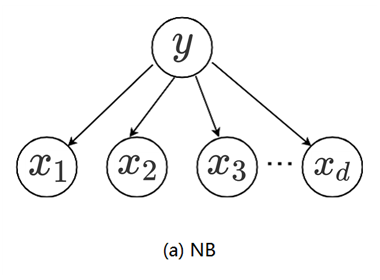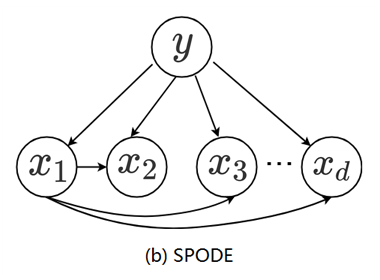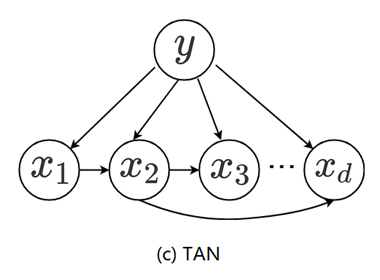图 1.1    朴素贝叶斯与两种半朴素贝叶斯分类器所考虑的属性依赖关系

TAN（Tree Augmented Naive Bayes）则是在最大带权生成树（maximum weighted spanning tree）算法的基础上，通过以下步骤将属性间依赖关系约简为如图 1.1（c）所示的树形结构：

计算任意两个属性之间的条件互信息（conditional mutual information）
$I(x_i,x_j|y) = \sum_{x_i,y_i;c\in\mathcal{Y}} P(x_i,x_j|c) \log \frac{P(x_i,x_j|c)}{P(x_i|c)P(x_j|c)} \tag{1.23}$

以属性为结点构建完全图，任意两个结点之间边的权重设为 $I(x_i,x_j|y)$。

构建此完全图的最大带权生成树，挑选根变量，将边置为有向。

加入类别结点 $y$，增加从 $y​$ 到每个属性的有向边。

容易看出，条件互信息 $I(x_i,x_j|y)$ 刻画了属性 $x_i$ 和 $x_j​$ 在已知类别情况下的相关性，因此，通过最大生成树算法，TAN 实际上仅保留了强相关属性之间的依赖性。
AODE（Averaged One-Dependent Estimator）是一种基于集成学习机制、更为强大的独依赖分类器。与 SPODE 通过模型选择确定超父属性不同，AODE 尝试将每个属性作为超父来构建 SPODE，然后将那些具有足够训练数据支撑的 SPODE 集成起来作为最终结果，即
$P(c|\mathbf{x}) \propto \sum_{i=1, \; |D_{x_i}|\geq m^{'}}^{n} P(c,x_i) \prod_{j=1}^{n}P(x_j | c,x_i) \tag{1.24}$
其中 $D_{x_i}$ 是在第 $i$ 个属性上取值为 $x_i$ 的样本的集合，$m^{'}$ 为阈值常数（一般默认设置为 30）。显然 AODE 需估计  $P(c,x_i)$ 和 $P(x_j|c,x_i)$。类似式（7.20），有
$\hat{P}(c,x_i) = \frac{|D_{c,x_i}|+1}{|D|+N_i} \tag{1.25}$
$\hat{P}(x_j|c,x_i) = \frac{|D_{c,x_i,x_j}|+1}{|D_{c,x_i}|+N_j} \tag{1.26}$
其中 $N_i$ 是第 $i$ 个属性可能的取值数量，$D_{c,x_i}$ 是类别为 $c$ 且在第 $i$ 个属性上取值为 $x_i$ 的样本集合，$D_{c,x_i,x_j}$ 是类别为 $c$ 且在第 $i$ 个和第 $j$ 个属性上取值分别为 $x_i$ 和 $x_j​$ 的样本集合。
不难看出，与朴素贝叶斯分类器类似，AODE 的训练过程也是“计数”，即在训练数据集上对符合条件的样本进行计数的过程。与朴素贝叶斯分类器相似，AODE 无需模型选择，即能通过预计算节省预测时间，也能采取懒惰学习方式在预测时再进行计数，并且易于实现增量学习。
既然将属性条件独立性假设放松为独依赖假设可能获得泛化性能的提升，那么，能否通过考虑属性间的高阶依赖（即对多个属性依赖）来进一步提升泛化性能呢？也就是说，将式（1.22）中的属性 $pa_i​$ 替换为包含 $k​$ 个属性的集合 $\mathbf{pa_i}​$，从而将 ODE 拓展为 $k​$DE。需注意的是，随着 $k​$ 的增加，准确估计概率 $P(x_i |y, \mathbf{pa_i})​$ 所需的训练样本数量将以指数级增加。因此，若训练数据非常充分，泛化性能有可能提升；但在有限样本条件下，则又陷入估计高阶联合概率的泥沼。

参考：
 周志华《机器学习》
 李航《统计学习方法》
 算法杂货铺——分类算法之朴素贝叶斯分类(Naive Bayesian classification)


展开全文贝叶斯定理 贝叶斯决策论
•算法 人工智能
• 贝叶斯分类器 学习讲义贝叶斯分类器 学习讲义贝叶斯分类器 学习讲义贝叶斯分类器 学习讲义贝叶斯分类器 学习讲义贝叶斯分类器 学习讲义...